## A Birthday Present for Fido

ejolson
Posts: 10725
Joined: Tue Mar 18, 2014 11:47 am

### Re: A Birthday Present for Fido

ejolson wrote:
Sun May 30, 2021 6:08 am
Another thing I like about Jack's version of Super Wumpus is that one doesn't hunt to kill, but instead uses immobilizing darts to tame it. This seems to anticipate the greater environmental awareness needed in today's world when dealing with Wumpi and other endangered species.
From what I can tell, the wumpus is no longer on the endangered species list. In fact, entire herds of wumpi have been spotted on the Raspberry Pico at

viewtopic.php?p=1893206#p1893206

as well as

viewtopic.php?p=1864167#p1864167

Another type of wumpus was also found recently on the Pico at

https://blog.smittytone.net/2021/02/20/ ... ico-style/

viewtopic.php?p=1890359#p1890359

ejolson
Posts: 10725
Joined: Tue Mar 18, 2014 11:47 am

### Re: A Birthday Present for Fido

ejolson wrote:
Mon Jul 26, 2021 7:05 am
ejolson wrote:
Sun May 30, 2021 6:08 am
Another thing I like about Jack's version of Super Wumpus is that one doesn't hunt to kill, but instead uses immobilizing darts to tame it. This seems to anticipate the greater environmental awareness needed in today's world when dealing with Wumpi and other endangered species.
From what I can tell, the wumpus is no longer on the endangered species list. In fact, entire herds of wumpi have been spotted on the Raspberry Pico at

viewtopic.php?p=1893206#p1893206

as well as

viewtopic.php?p=1864167#p1864167

Another type of wumpus was also found recently on the Pico at

https://blog.smittytone.net/2021/02/20/ ... ico-style/

viewtopic.php?p=1890359#p1890359
The port of BBC Basic to the Raspberry Pico now works well enough for wumpus hunting. In particular, an adapted version of Gregory Yob's original code is now one of the example programs that run on Pico.

http://fractal.math.unr.edu/~ejolson/pi ... system.uf2

http://fractal.math.unr.edu/~ejolson/pi/pico/extra.zip

ejolson
Posts: 10725
Joined: Tue Mar 18, 2014 11:47 am

### Re: A Birthday Present for Fido

ejolson wrote:
Fri May 28, 2021 3:10 am
Looking for dodecahedrons that appear or disappear when one version of rdice is used in place of the other seems similar to mining for Bitcoin, especially now that one can't buy a Tesla using the latter.
According to the birthday dog, every computation can be translated into a problem in graph theory. While the theoretical implications of such statements are usually focused on, here is a program which computes Vornonoi tessellations of a periodic grid using a parallel form of Dijkstra's algorithm:

Code: Select all

``````/*  voronoi--Parallel Voronoi Tessellator
Written January 2022 by Eric Olson */

package main

import("fmt"; "runtime"; "os"; "bufio"; "time"
"encoding/binary"; "sync/atomic")

const (N=512; Nobs=128)

var tictime float64
func tic() {
now:=time.Now()
tictime=float64(now.Unix())+1.0E-9*float64(now.Nanosecond())
}
func toc() float64 {
now:=time.Now()
return float64(now.Unix())+1.0E-9*float64(now.Nanosecond())-tictime
}

type wmsgen struct {
x,w,s uint64
}
func ipoly8(x uint64) uint64 {
x2:=x*x
return (((x2*x2+
0x4cca10b00bc5da27)*x2+
0x872a4954ca3a3537)*x+
0x2bf0e3ddcc4fb44f)*x+
}
func (p *wmsgen)rint32() uint32 {
p.x*=p.x; p.w+=p.s
p.x+=p.w; p.x=(p.x>>32)|(p.x<<32)
return uint32(p.x)
}
func (p *wmsgen)rfloat() float64 {
return float64(p.rint32())/(1<<32)
}
func (p *wmsgen)rdice(n int) int {
d:=uint32(1<<32/uint64(n))
for {
r:=int(p.rint32()/d)
if r<n {
return r
}
}
}
func mkwsgen(x uint64) wmsgen {
var p wmsgen
p.x=x; p.w=0
p.s=ipoly8(x)|1
return p
}

type gpspec struct {
i,j,c,d int
}

var (grid [N][N]gpspec; obs [Nobs]gpspec)
var heap [][]gpspec
var hchan []chan gpspec
var dchan chan int
var cdone int32

type rgbtype struct {
r,g,b byte
}

func wrgrid(){
for i:=0;i<N;i++ {
for j:=0;j<N;j++ {
if grid[i][j].c<0 {
fmt.Printf("*")
} else {
fmt.Printf("%d",grid[i][j].c)
}
}
fmt.Printf("\n")
}
}

func prgrid(){
p:=mkwsgen(791)
io,_:=os.Create("chg.ppm")
fp:=bufio.NewWriter(io)
fmt.Fprintf(fp,"P6 %d %d 255\n",N,N)
var (colors [Nobs]rgbtype; black rgbtype)
for k:=0;k<Nobs;k++ {
colors[k].r=byte(p.rdice(256))
colors[k].g=byte(p.rdice(256))
colors[k].b=byte(p.rdice(256))
}
for i:=0;i<N;i++ {
var raster [N]rgbtype
for j:=0;j<N;j++ {
if grid[i][j].d<=6||grid[i][j].c<0 {
raster[j]=black
} else {
raster[j]=colors[grid[i][j].c]
}
}
binary.Write(fp,binary.LittleEndian,raster)
}
fp.Flush()
io.Close()
}

func insertgp(hp *[]gpspec,p gpspec){
var r,s int
r=len(*hp)
*hp=append(*hp,p)
for {
s=(r-1)/2
if s==r { return }
if (*hp)[r].d>=(*hp)[s].d { return }
(*hp)[s],(*hp)[r]=(*hp)[r],(*hp)[s]
r=s
}
}

func extractgp(hp *[]gpspec)gpspec {
var r0,r1,s0,s1 int
if len(*hp)==0 {
fmt.Printf("Tried to remove nonexistent point!")
os.Exit(1)
}
p:=(*hp)
r0=len(*hp)-1; s0=0
(*hp)[s0]=(*hp)[r0]; *hp=(*hp)[:r0]
for {
r0=2*s0+1; r1=r0+1
if r0>=len(*hp) { return p }
s1=r0
if r1<len(*hp) {
if (*hp)[r0].d>=(*hp)[r1].d { s1=r1 }
}
if (*hp)[s1].d>=(*hp)[s0].d { return p }
(*hp)[s0],(*hp)[s1]=(*hp)[s1],(*hp)[s0]
s0=s1
}
}

func dist1(i1,i2 int)int {
di:=i1-i2
if di>N/2 { di-=N
} else if di<=-N/2 { di+=N }
return di
}

func dist2(p1,p2 gpspec)int {
di:=dist1(p1.i,p2.i)
dj:=dist1(p1.j,p2.j)
return di*di+dj*dj
}

func mkobs(p *wmsgen){
for i:=0;i<N;i++ {
for j:=0;j<N;j++ {
grid[i][j].i=i
grid[i][j].j=j
grid[i][j].c=-2147483648
grid[i][j].d=2147483647
}
}
for k:=0;k<Nobs;k++ {
i:=p.rdice(N)
j:=p.rdice(N)
obs[k].i=i; obs[k].j=j
obs[k].d=0; obs[k].c=k
}
}

func ijtoc(i,j int)int {
im:=i*mdiv/N; jm:=j*ndiv/N
return im*ndiv+jm
}

func gogrid(p int){
for {
if len(hchan[p])>0 {
gp:=<-hchan[p]
if gp.c<0 {
dchan<-p
return
}
insertgp(&heap[p],gp)
} else if len(heap[p])>0 {
gp:=extractgp(&heap[p])
i:=gp.i; j:=gp.j; c:=gp.c
if grid[i][j].d<gp.d { continue }
grid[i][j]=gp
for di:=-1;di<=1;di++ {
for dj:=-1;dj<=1;dj++ {
if di==0&&dj==0 { continue }
i1:=(N+i+di)%N; j1:=(N+j+dj)%N
d:=dist2(grid[i1][j1],obs[c])
if d<grid[i1][j1].d {
p2:=ijtoc(i1,j1)
if p==p2 {
grid[i1][j1].c=c
grid[i1][j1].d=d
insertgp(&heap[p],grid[i1][j1])
} else {
gp:=gpspec{i1,j1,c,d}
hchan[p2]<-gp
}
}
}
}
} else {
if p2==p { continue }
hchan[p2]<-gpspec{0,0,-1,0}
}
dchan<-p
return
}
gp:=<-hchan[p]
if gp.c<0 {
dchan<-p
return
}
insertgp(&heap[p],gp)

}
}
}

func fixgrid(hp *[]gpspec){
for len(*hp)>0 {
gp:=extractgp(hp)
i:=gp.i; j:=gp.j; c:=gp.c
if grid[i][j].d<gp.d { continue }
for di:=-1;di<=1;di++ {
for dj:=-1;dj<=1;dj++ {
if di==0&&dj==0 { continue }
i1:=(N+i+di)%N; j1:=(N+j+dj)%N
d:=dist2(grid[i1][j1],obs[c])
if d<grid[i1][j1].d {
grid[i1][j1].c=c
grid[i1][j1].d=d
insertgp(hp,grid[i1][j1])
}
}
}
}
}

func mkgrid(){
heap[nt]=heap[nt][:0]
}
for k:=0;k<Nobs;k++ {
i:=obs[k].i; j:=obs[k].j
grid[i][j]=obs[k]
nt:=ijtoc(i,j)
insertgp(&heap[nt],grid[i][j])
}
cdone=0
go gogrid(nt)
}
gogrid(0)
smax:=0; rt:=0
r:=<-dchan
s:=len(heap[r])+len(hchan[r])
if smax<s { smax=s; rt=r }
}
if smax>0 {
// The race left something in the queues
if nt==rt { continue }
for len(hchan[nt])>0 {
gp:=<-hchan[nt]
if gp.c<0 { continue }
insertgp(&heap[rt],gp)
}
for k:=0;k<len(heap[nt]);k++ {
insertgp(&heap[rt],heap[nt][k])
}
}
fixgrid(&heap[rt])
}
}

func getpart()(int,int){
m:=1; n:=1
for {
if m*n>=ncpu { return m,n }
m++
if m*n>=ncpu { return m,n }
n++
}
}

func main(){
ncpu=runtime.GOMAXPROCS(0)
fmt.Printf("voronoi--Parallel Voronoi Tessellator\n"+
"Written 2022 by Eric Olson (GOMAXPROCS=%d)\n\n",ncpu)
tic(); ticstart:=tictime
fmt.Printf("%10s %10s %11s %7s %9s %12s\n",
for mmax:=1;mmax<=12;mmax++ {
mdiv=mmax; ndiv=mmax
heap[c]=make([]gpspec,0,N*Nobs)
}
fmt.Printf("%10s %10d %11s %7d %9d ",
fmt.Sprintf("%dx%d",N,N), Nobs,
fmt.Sprintf("%dx%d",mdiv,ndiv),
p:=mkwsgen(135713)
var t float64
Niter:=1
for {
tic()
for k:=0;k<Niter;k++ {
mkobs(&p)
mkgrid()
}
t=toc()
if t>5 {
break
}
Niter*=2
}
fmt.Printf("%12.4f\n",float64(Niter)/t)
}
prgrid()
tictime=ticstart
fmt.Printf("\nTotal execution time %g seconds.\n",toc())
os.Exit(0)
}
``````
Note this is a parallel Go version of the C code which appeared in

viewtopic.php?p=1962396#p1962396

The program partitions a 512x512 grid representing a graph with 262144 vertices into a number of subgraphs and performs the Dijkstra algorithm in parallel on each subgraph using Go channels to transfer minimal path information between subgraphs as needed.

As shown by the Pi4 runs

Code: Select all

``````\$ ./voronop
voronoi--Parallel Voronoi Tessellator
Written 2022 by Eric Olson (GOMAXPROCS=4)

Grid   Polygons   Partition   Cores   Threads         Rate
512x512        128         1x1       4         1       5.6078
512x512        128         2x2       4         4      16.7401
512x512        128         3x3       4         9      18.8761
512x512        128         4x4       4        16      21.4690
512x512        128         5x5       4        25      22.1106
512x512        128         6x6       4        36      22.6328
512x512        128         7x7       4        49      22.3989
512x512        128         8x8       4        64      22.0039
512x512        128         9x9       4        81      21.6141
512x512        128       10x10       4       100      20.9502
512x512        128       11x11       4       121      20.5593
512x512        128       12x12       4       144      17.8982

Total execution time 149.4959921836853 seconds.
``````
and

Code: Select all

``````\$ taskset -c 0 ./voronop
voronoi--Parallel Voronoi Tessellator
Written 2022 by Eric Olson (GOMAXPROCS=1)

Grid   Polygons   Partition   Cores   Threads         Rate
512x512        128         1x1       1         1       5.5686
512x512        128         2x2       1         4       6.6372
512x512        128         3x3       1         9       6.5456
512x512        128         4x4       1        16       6.3399
512x512        128         5x5       1        25       6.1819
512x512        128         6x6       1        36       6.0230
512x512        128         7x7       1        49       5.8274
512x512        128         8x8       1        64       5.6392
512x512        128         9x9       1        81       5.4943
512x512        128       10x10       1       100       5.3404
512x512        128       11x11       1       121       5.1906
512x512        128       12x12       1       144       5.0209

Total execution time 151.51451539993286 seconds.
``````
Since

22.6328/6.6372=3.4

one obtains good parallel scaling when moving from one to four cores on a Pi 4B. Unfortunately, results are not so impressive when moving to more cores on an x86 system, perhaps due to locking overhead in the Go channels.

For reference, here is a typical Voronoi tessellation:chg.png (21 KiB) Viewed 3905 times
When I showed my results to Fido the only comment was on the poorness of the uniform distribution obtained from the Weyl middle-square random number generator. If the randomness is good enough for Hunt the Wumpus, my claim is that it should also be more than enough for everything else.
Last edited by ejolson on Fri Jan 21, 2022 5:56 am, edited 1 time in total.

ejolson
Posts: 10725
Joined: Tue Mar 18, 2014 11:47 am

### Re: A Birthday Present for Fido

ejolson wrote:
Wed Jan 19, 2022 8:35 pm
When I showed my results to Fido the only comment was on the poorness of the uniform distribution obtained from the Weyl middle-square random number generator. If the randomness is good enough for Hunt the Wumpus, my claim is that it should also be more than enough for everything else.
The head of quality assurance and dog treats just issued a scathing analysis of my Go code: Don't flood the priority queue with diagonal moves--programming is not politics. After an order of free government-issue face masks and a few simple changes to the program, I obtained

Code: Select all

``````\$ ./voronop
voronoi--Parallel Voronoi Tessellator
Written 2022 by Eric Olson (GOMAXPROCS=4)

Grid   Polygons   Partition   Cores   Threads         Rate
512x512        128         1x1       4         1       6.8263
512x512        128         2x2       4         4      19.0319
512x512        128         3x3       4         9      23.3825
512x512        128         4x4       4        16      27.0344
512x512        128         5x5       4        25      28.7413
512x512        128         6x6       4        36      30.0660
512x512        128         7x7       4        49      30.4874
512x512        128         8x8       4        64      30.8746
512x512        128         9x9       4        81      30.5655
512x512        128       10x10       4       100      30.4512
512x512        128       11x11       4       121      29.7786
512x512        128       12x12       4       144      29.7882

Total execution time 200.4169111251831 seconds.
``````
and

Code: Select all

``````\$ taskset -c 0 ./voronop
voronoi--Parallel Voronoi Tessellator
Written 2022 by Eric Olson (GOMAXPROCS=1)

Grid   Polygons   Partition   Cores   Threads         Rate
512x512        128         1x1       1         1       6.6713
512x512        128         2x2       1         4       8.0008
512x512        128         3x3       1         9       8.1564
512x512        128         4x4       1        16       8.3443
512x512        128         5x5       1        25       8.3682
512x512        128         6x6       1        36       8.2862
512x512        128         7x7       1        49       8.2545
512x512        128         8x8       1        64       8.1244
512x512        128         9x9       1        81       8.0024
512x512        128       10x10       1       100       7.8729
512x512        128       11x11       1       121       7.7150
512x512        128       12x12       1       144       7.6106

Total execution time 193.9240427017212 seconds.
``````
on a Pi 4B running at 1500 MHz. As

30.8746 / 22.6328 = 1.36

and

8.3682 / 6.6372 = 1.26

not only is the new program 30 percent faster, but the parallel scaling of 3.7 using four cores is also better.

For reference the code is

Code: Select all

``````/*  voronoi--Parallel Voronoi Tessellator
Written January 2022 by Eric Olson

Removed diagonal links and first reflections
Resolve ties in distance deterministically */

package main

import("fmt"; "runtime"; "os"; "bufio"; "time"
"encoding/binary"; "sync/atomic")

const (N=512; Nobs=128)

var tictime float64
func tic() {
now:=time.Now()
tictime=float64(now.Unix())+1.0E-9*float64(now.Nanosecond())
}
func toc() float64 {
now:=time.Now()
return float64(now.Unix())+1.0E-9*float64(now.Nanosecond())-tictime
}

type wmsgen struct {
x,w,s uint64
}
func ipoly8(x uint64) uint64 {
x2:=x*x
return (((x2*x2+
0x4cca10b00bc5da27)*x2+
0x872a4954ca3a3537)*x+
0x2bf0e3ddcc4fb44f)*x+
}
func (p *wmsgen)rint32() uint32 {
p.x*=p.x; p.w+=p.s
p.x+=p.w; p.x=(p.x>>32)|(p.x<<32)
return uint32(p.x)
}
func (p *wmsgen)rfloat() float64 {
return float64(p.rint32())/(1<<32)
}
func (p *wmsgen)rdice(n int) int {
d:=uint32(1<<32/uint64(n))
for {
r:=int(p.rint32()/d)
if r<n {
return r
}
}
}
func mkwsgen(x uint64) wmsgen {
var p wmsgen
p.x=x; p.w=0
p.s=ipoly8(x)|1
return p
}

type gpspec struct {
i,j,c,d int
}
type dirspec struct {
i,j int
}

var (grid [N][N]gpspec; obs [Nobs]gpspec)
var heap [][]gpspec
var origs [][Nobs]bool
var hchan []chan gpspec
var dchan chan int
var cdone int32
var dirs=dirspec{
dirspec{-1,0},dirspec{0,-1},dirspec{1,0},dirspec{0,1}}

type rgbtype struct {
r,g,b byte
}

func wrgrid(){
for i:=0;i<N;i++ {
for j:=0;j<N;j++ {
if grid[i][j].c<0 {
fmt.Printf("*")
} else {
fmt.Printf("%d",grid[i][j].c)
}
}
fmt.Printf("\n")
}
}

func prgrid(){
p:=mkwsgen(791)
io,_:=os.Create("chg.ppm")
fp:=bufio.NewWriter(io)
fmt.Fprintf(fp,"P6 %d %d 255\n",N,N)
var (colors [Nobs]rgbtype; black rgbtype)
for k:=0;k<Nobs;k++ {
colors[k].r=byte(p.rdice(256))
colors[k].g=byte(p.rdice(256))
colors[k].b=byte(p.rdice(256))
}
for i:=0;i<N;i++ {
var raster [N]rgbtype
for j:=0;j<N;j++ {
if grid[i][j].d<=6||grid[i][j].c<0 {
raster[j]=black
} else {
raster[j]=colors[grid[i][j].c]
}
}
binary.Write(fp,binary.LittleEndian,raster)
}
fp.Flush()
io.Close()
}

func insertgp(hp *[]gpspec,p gpspec){
var r,s int
r=len(*hp)
*hp=append(*hp,p)
for {
s=(r-1)/2
if s==r { return }
if (*hp)[r].d>=(*hp)[s].d { return }
(*hp)[s],(*hp)[r]=(*hp)[r],(*hp)[s]
r=s
}
}

func extractgp(hp *[]gpspec)gpspec {
var r0,r1,s0,s1 int
if len(*hp)==0 {
fmt.Printf("Tried to remove nonexistent point!")
os.Exit(1)
}
p:=(*hp)
r0=len(*hp)-1; s0=0
(*hp)[s0]=(*hp)[r0]; *hp=(*hp)[:r0]
for {
r0=2*s0+1; r1=r0+1
if r0>=len(*hp) { return p }
s1=r0
if r1<len(*hp) {
if (*hp)[r0].d>=(*hp)[r1].d { s1=r1 }
}
if (*hp)[s1].d>=(*hp)[s0].d { return p }
(*hp)[s0],(*hp)[s1]=(*hp)[s1],(*hp)[s0]
s0=s1
}
}

func dist1(i1,i2 int)int {
di:=i1-i2
if di>N/2 { di-=N
} else if di<=-N/2 { di+=N }
return di
}

func dist2(p1,p2 gpspec)int {
di:=dist1(p1.i,p2.i)
dj:=dist1(p1.j,p2.j)
return di*di+dj*dj
}

func mkobs(p *wmsgen){
for i:=0;i<N;i++ {
for j:=0;j<N;j++ {
grid[i][j].i=i
grid[i][j].j=j
grid[i][j].c=-2147483648
grid[i][j].d=2147483647
}
}
for k:=0;k<Nobs;k++ {
i:=p.rdice(N)
j:=p.rdice(N)
obs[k].i=i; obs[k].j=j
obs[k].d=0; obs[k].c=k
}
}

func ijtoc(i,j int)int {
im:=i*mdiv/N; jm:=j*ndiv/N
return im*ndiv+jm
}

func gogrid(p int){
for {
if len(hchan[p])>0 {
gp:=<-hchan[p]
if gp.c<0 {
dchan<-p
return
}
insertgp(&heap[p],gp)
} else if len(heap[p])>0 {
gp:=extractgp(&heap[p])
i:=gp.i; j:=gp.j
if grid[i][j].d<gp.d { continue }
if grid[i][j].d==gp.d {
if grid[i][j].c<gp.c {
grid[i][j].c=gp.c
} else {
gp.c=grid[i][j].c
}
} else {
grid[i][j].c=gp.c
grid[i][j].d=gp.d
}
c:=gp.c
for d:=0;d<4;d++ {
di:=dirs[d].i; dj:=dirs[d].j
i1:=(N+i+di)%N; j1:=(N+j+dj)%N
d:=dist2(grid[i1][j1],obs[c])
if d<grid[i1][j1].d {
p2:=ijtoc(i1,j1)
if p==p2 {
grid[i1][j1].c=c
grid[i1][j1].d=d
insertgp(&heap[p],grid[i1][j1])
} else {
if !origs[p2][c] {
gp:=gpspec{i1,j1,c,d}
hchan[p2]<-gp
}
}
} else if d==grid[i1][j1].d {
if grid[i1][j1].c<gp.c {
grid[i1][j1].c=c
//                      insertgp(&heap[p],grid[i1][j1])
}
}
}
} else {
if p2==p { continue }
hchan[p2]<-gpspec{0,0,-1,0}
}
dchan<-p
return
}
gp:=<-hchan[p]
if gp.c<0 {
dchan<-p
return
}
insertgp(&heap[p],gp)
}
}
}

func fixgrid(hp *[]gpspec){
for len(*hp)>0 {
gp:=extractgp(hp)
i:=gp.i; j:=gp.j
if grid[i][j].d<gp.d { continue }
if grid[i][j].d==gp.d {
if grid[i][j].c<gp.c {
grid[i][j].c=gp.c
} else {
gp.c=grid[i][j].c
}
} else {
grid[i][j].c=gp.c
grid[i][j].d=gp.d
}
c:=gp.c
for d:=0;d<len(dirs);d++ {
di:=dirs[d].i; dj:=dirs[d].j
i1:=(N+i+di)%N; j1:=(N+j+dj)%N
d:=dist2(grid[i1][j1],obs[c])
if d<grid[i1][j1].d {
grid[i1][j1].c=c
grid[i1][j1].d=d
insertgp(hp,grid[i1][j1])
} else if d==grid[i1][j1].d {
if grid[i1][j1].c<gp.c {
grid[i1][j1].c=c
//                  insertgp(hp,grid[i1][j1])
}
}
}
}
}

func mkgrid(){
heap[nt]=heap[nt][:0]
for k:=0;k<Nobs;k++ { origs[nt][k]=false }
}
for k:=0;k<Nobs;k++ {
i:=obs[k].i; j:=obs[k].j
grid[i][j]=obs[k]
nt:=ijtoc(i,j)
origs[nt][k]=true
insertgp(&heap[nt],grid[i][j])
}
cdone=0
go gogrid(nt)
}
gogrid(0)
smax:=0; rt:=0
r:=<-dchan
s:=len(heap[r])+len(hchan[r])
if smax<s { smax=s; rt=r }
}
if smax>0 {
if nt==rt { continue }
for len(hchan[nt])>0 {
gp:=<-hchan[nt]
if gp.c<0 { continue }
insertgp(&heap[rt],gp)
}
for k:=0;k<len(heap[nt]);k++ {
insertgp(&heap[rt],heap[nt][k])
}
}
fixgrid(&heap[rt])
}
}

func getpart()(int,int){
m:=1; n:=1
for {
if m*n>=ncpu { return m,n }
m++
if m*n>=ncpu { return m,n }
n++
}
}

func main(){
ncpu=runtime.GOMAXPROCS(0)
fmt.Printf("voronoi--Parallel Voronoi Tessellator\n"+
"Written 2022 by Eric Olson (GOMAXPROCS=%d)\n\n",ncpu)
tic(); ticstart:=tictime
fmt.Printf("%10s %10s %11s %7s %9s %12s\n",
for mmax:=1;mmax<=12;mmax++ {
mdiv=mmax; ndiv=mmax
heap[c]=make([]gpspec,0,N*Nobs)
}
fmt.Printf("%10s %10d %11s %7d %9d ",
fmt.Sprintf("%dx%d",N,N), Nobs,
fmt.Sprintf("%dx%d",mdiv,ndiv),
p:=mkwsgen(135713)
var t float64
Niter:=1
for {
tic()
for k:=0;k<Niter;k++ {
mkobs(&p)
mkgrid()
}
t=toc()
if t>5 {
break
}
Niter*=2
}
fmt.Printf("%12.4f\n",float64(Niter)/t)
}
prgrid()
tictime=ticstart
fmt.Printf("\nTotal execution time %g seconds.\n",toc())
os.Exit(0)
}
``````

ejolson
Posts: 10725
Joined: Tue Mar 18, 2014 11:47 am

### Re: A Birthday Present for Fido

ejolson wrote:
Fri Jan 21, 2022 1:15 am
ejolson wrote:
Wed Jan 19, 2022 8:35 pm
When I showed my results to Fido the only comment was on the poorness of the uniform distribution obtained from the Weyl middle-square random number generator. If the randomness is good enough for Hunt the Wumpus, my claim is that it should also be more than enough for everything else.
The head of quality assurance and dog treats just issued a scathing analysis of my Go code: Don't flood the priority queue with diagonal moves--programming is not politics.
Another year has passed and the dog developer is having another birthday. My plan is to create an optimal AI based on Bayesian statistics for playing the battleship game described as SALVO in David Ahl's classic 101 Basic Computer Games where
Larry Siegel wrote: SALVO is played on a 10x10 grid or board using an x,y coordinate system. The player has 4 ships: battleship (5 squares), cruiser (3 squares), and two destroyers (2 squares each). The ships must be placed horizontally, vertically, or diagonally and must not overlap. The ships do not move during the game.

As long as any square of a battleship still survives, the player is allowed three shots, for a cruiser 2 shots, and for each destroyer 1 shot. Thus, at the beginning of the game the player has 3+2+1+1=7 shots. The player enters all of his shots and the computer tells what was hit. II A shot is entered by its grid coordinates, x,y. The winner is the one who sinks all of the opponent's ships.
http://www.bitsavers.org/pdf/dec/_Books ... _Mar75.pdf

Note a Milton Bradly game with the same theme was marketed using the famous line "you sank my battleship" as heard in the advertisement

This is a simplified version of SALVO. An even simpler version of the game was recently finished in the Black Sea, though it's not clear who sank the battleship.

In 2013 and 2014 a Pi versus Pi battleship tournament was part of the Blue Pi Thinking program at the University of York. In particular,
Gordon Hollingworth wrote: BattlePi is a game of battleships played automatically by the Raspberry Pi with a server through which two Raspberry Pis can communicate. The students are given the (well commented Python) software which initially just chooses random positions to make shots at. The student then has to modify the python code to implement better AI in both firing position and ship placement.
https://www.raspberrypi.com/news/let-th ... -commence/

https://www.raspberrypi.com/news/blue-p ... y-of-york/

Before computing the Bayesian posterior after a salvo of Neptune cruise missiles, the first part of Fido's new birthday project will be to strategically place the ships in a statistically unbiased way and then develop a framework that allows the super pet to play against other computers. I'm personally looking forward to making an improved version of the program I wrote long ago for the Apple II.

ejolson
Posts: 10725
Joined: Tue Mar 18, 2014 11:47 am

### Re: A Birthday Present for Fido

ejolson wrote:
Sat Apr 16, 2022 3:31 am
the first part of Fido's new birthday project will be to strategically place the ships in a statistically unbiased way and then develop a framework that allows the super pet to play against other computers.
Ideally at the beginning of the game the chances that a ship occupies any of the 100 cells in a 10x10 grid should be equal. Anything else would provide the attacker with information about where the ship is before firing even one cruise missile.

Unfortunately, if the location and heading of the battleship is simply chosen at random, then the fact that there are only 3 ways a ship of length five can occupy a corner is dwarfed by the 20 different ways it can occupy a square in the center. Moreover, since any ship which fits in a corner also occupies other cells, it's not clear it's even possible to construct a ship placement algorithm that is unbiased.

While yesterday's newshttps://www.foxnews.com/us/uss-sullivan ... ks-buffalo

indicates that it really is possible for a battleship to sink on its own, it makes sense to position the boat in a way that hides it from missiles.

The plan then is to test different ways to randomly position the boats and then use a Monte Carlo technique to check if the resulting probability that any particular cell on the 10x10 grid contains a boat is approximately uniform.

ejolson
Posts: 10725
Joined: Tue Mar 18, 2014 11:47 am

### Re: A Birthday Present for Fido

ejolson wrote:
Sat Apr 16, 2022 3:31 am
I'm personally looking forward to making an improved version of the program I wrote long ago for the Apple II.
It doesn't look like I'll find that Apple II program; however, I did find a Fortran program from 1983 with a comment that indicates I wrote it. It starts out like

Code: Select all

``````C
C      BATTLESHIP  V2.0
C      BY ERIC OLSON
C      JUNE 6, 1983
C
PROGRAM BATTLE
INTEGER*2 US(10,10), THEM(10,10), LENGTH(5), RECORD(5,5)
INTEGER*2 X, Y, Z, X1, Y1, DIRECT(8,2), COUNT, SHOTS(5), ROUND
INTEGER*2 SHOOT, USREC(5), THINK(10,10), FIND(5), POSSIB
INTEGER*2 FIRE(2,20), HIT(5), MISS, TURN
INTEGER*2 H, V, B, L1, L2, L3, I, J, K
INTEGER*4 SEED
REAL*4 CHANCE(10,10), PERCNT, RAND
LOGICAL*2 USHIPS(5), THIPS(5), REV, DEBUG, KLUDGE
DATA DIRECT/0,-1,-1,-1,0,1,1,1,1,1,0,-1,-1,-1,0,1/
DATA LENGTH/5,3,2,2,0/
DATA US/100*0/, THEM/100*0/, SHOTS/3,2,1,1,0/
C
C      SET RUN OPTIONS
C
``````
and then continues in the style of Fortran 66 for about 500 lines. Given it is marked at version 2.0, it must be a rewrite of the Apple program.

While the trip down memory lane is nice--especially during this modern time of war, famine and epidemic--what's more important is that it's again clear ships can be placed diagonally in this version of battleship, unlike the Milton Bradly game but just like the SALVO program from 101 Basic Computer Games.

On the other hand, it appears the ship placement routine in the Fortran code, allows diagonal ships to intersect each other, which certainly shouldn't be permitted. It also doesn't make any attempts to place the ships so the chances of any cell in the grid being occupied has equal probability.

It's tempting to see how far I get with gfortran compiling that code; however, the dog developer recommends a complete rewrite in a modern programming language. Even though FidoBasic is still not available, I'm hoping it will be possible to write a better program after 39 years.
Last edited by ejolson on Sun Apr 17, 2022 4:24 pm, edited 2 times in total.

jahboater
Posts: 8608
Joined: Wed Feb 04, 2015 6:38 pm
Location: Wonderful West Dorset

### Re: A Birthday Present for Fido

ejolson wrote:
Sun Apr 17, 2022 6:57 am
It's tempting to see how far I get with gfortran compiling that code; however, the dog developer recommends a complete rewrite in a modern programming language.
Or in Fortran 2018 which does seem modern, and would not require a complete rewrite.

also -ffree-form and "implicit none"

The good vectorization in Fortran, or -fopenmp, or just whole array stuff might help with an exhaustive search for ships.

ejolson
Posts: 10725
Joined: Tue Mar 18, 2014 11:47 am

### Re: A Birthday Present for Fido

jahboater wrote:
Sun Apr 17, 2022 9:12 am
ejolson wrote:
Sun Apr 17, 2022 6:57 am
It's tempting to see how far I get with gfortran compiling that code; however, the dog developer recommends a complete rewrite in a modern programming language.
Or in Fortran 2018 which does seem modern, and would not require a complete rewrite.

also -ffree-form and "implicit none"

The good vectorization in Fortran, or -fopenmp, or just whole array stuff might help with an exhaustive search for ships.
The flag -std=legacy reduced the number of errors down from about 400 to twelve. Those were easily remedied and I now have a program that works as well as it did back in 1983. It trounced me in the first game, even though I cheated and printed out where the enemy ships were about half way through.

I guess that shows even perfect military intelligence can't fully offset a competent command and especially make up for a fully incompetent one.

The code still resembles Fortran 66 and the user interface is lacking. After some cleanup I'll post a run and the source as a baseline for future improvement.

I wonder if Fido would like a York-style BattlePi competition at the birthday party. Since best out of three games doesn't seem statistically significant, maybe best out of 333 would make a better match.

ejolson
Posts: 10725
Joined: Tue Mar 18, 2014 11:47 am

### Re: A Birthday Present for Fido

ejolson wrote:
Sat Apr 16, 2022 3:31 am
My plan is to create an optimal AI based on Bayesian statistics for playing the battleship game described as SALVO in David Ahl's classic 101 Basic Computer Games

http://www.bitsavers.org/pdf/dec/_Books ... _Mar75.pdf
Arguably, Ahl leaving DEC in 1974 on account of differences of opinion with one of the founders of the company is how DEC missed the personal computer revolution, but never mind that.

While polishing my Fortran code, I decided to take a look at the original SALVO program as published in 101 Basic Computer Games to see if it really played by the same rules. It did. Then I thought, given the canine coder's affinity for Basic, why not convert the original program to run on the Raspberry Pi.

Searching online led to an electronic copy of the source

https://github.com/coding-horror/basic- ... /salvo.bas

Comparing the Github code with the version I typed in myself 40 years ago from the DEC book revealed two interesting differences:

Code: Select all

``````183c183
< 2800 IF B(X,Y)>10 THEN 2820
---
> 2800 IF A(X,Y)>10 THEN 2820
300c300
< 3970 K(R,S)=K(R,S)+E(U)-2*INT(H(U)+.5)
---
> 3970 K(R,S)=K(R,S)+E(U)-S*INT(H(U)+.5)
``````
These differences seem to be bugs introduced when Creative Computing republished SALVO in their Basic Computer Games Microcomputer Edition.

https://annarchive.com/files/Basic_Comp ... dition.pdf

It would appear the current race to the bottom began much earlier than I imagined and has now propagated to the code on Github. Do you think I should issue a pull request?

Since David Ahl was the founder of Creative Computing

https://en.wikipedia.org/wiki/David_H._Ahl

it's not surprising the games in the DEC publication were republished. Maybe it's not even surprising that whoever typed them in from the original book made a few typos in the process. I also found an error made from when I keyed in the same program 40 years ago which has now been fixed.

ejolson
Posts: 10725
Joined: Tue Mar 18, 2014 11:47 am

### Re: A Birthday Present for Fido

ejolson wrote:
Tue Apr 19, 2022 6:20 am
These differences seem to be bugs introduced when Creative Computing republished SALVO in their Basic Computer Games Microcomputer Edition.
I now have the Basic version of Salvo running. It even works on the Pico!

viewtopic.php?t=316761

I've been wondering how the typo on line 3970 in the microcomputer edition went undetected. Though it doesn't make sense to multiply the evaluation function by the column number S (at least to me), maybe it's a tricky improvement I don't understand. Unfortunately, none of the code which implements the computer's strategy makes much sense to me.

I played the game twice: Once with either version of line 3970. The game finished in both cases but I was trounced by the original version and won against the typo in the microcomputer edition. Since two runs is not enough to confirm which plays a stronger game, some sort of computer versus computer tournament might help.

Alternatively, could someone read the code and understand what it does?

Here is the code for BBC Basic:

Code: Select all

``````1000 REM *** SALVO BY LARRY SIEGEL
1010 REM *** LAST REVISION 6/9/73
1020 REM *** CHECKED OUT ON RSTS/E BY DAVE AHL, DIGITAL
1030 REM ***
1040 DIM A(10,10),B(10,10),C(7),D(7),E(12),F(12),G(12),H(12),K(10,10)
1050 Z8=0
1060 FOR W=1 TO 12
1070   E(W)=-1
1080   H(W)=-1
1090 NEXT W
1100 FOR X=1 TO 10
1110   FOR Y=1 TO 10
1120     B(X,Y)=0
1130   NEXT Y
1140 NEXT X
1150 FOR X=1 TO 12
1160   F(X)=0
1170   G(X)=0
1180 NEXT X
1190 FOR X=1 TO 10
1200   FOR Y=1 TO 10
1210     A(X,Y)=0
1220   NEXT Y
1230 NEXT X
1240 FOR K=4 TO 1 STEP -1
1250   U6=0
1260   GOSUB 2910
1270   DEF FNA(K)=(5-K)*3-2*INT(K/4)+SGN(K-1)-1
1280   DEF FNB(K)=K+INT(K/4)-SGN(K-1)
1290   IF V+V2+V*V2=0 THEN 1260
1300   IF Y+V*FNB(K)>10 THEN 1260
1310   IF Y+V*FNB(K)<1 THEN 1260
1320   IF X+V2*FNB(K)>10 THEN 1260
1330   IF X+V2*FNB(K)<1 THEN 1260
1340   U6=U6+1
1350   IF U6>25 THEN 1190
1360   FOR Z=0 TO FNB(K)
1370     F(Z+FNA(K))=X+V2*Z
1380     G(Z+FNA(K))=Y+V*Z
1390   NEXT Z
1400   U8=FNA(K)
1405   IF U8>U8+FNB(K) THEN 1460
1410   FOR Z2=U8 TO U8+FNB(K)
1415     IF U8<2 THEN 1450
1420     FOR Z3=1 TO U8-1
1430       IF SQR((F(Z3)-F(Z2))^2+(G(Z3)-G(Z2))^2)<3.59 THEN 1260
1440     NEXT Z3
1450   NEXT Z2
1460   FOR Z=0 TO FNB(K)
1470     A(F(Z+U8),G(Z+U8))=.5+SGN(K-1)*(K-1.5)
1480   NEXT Z
1490 NEXT K
1500 PRINT "ENTER COORDINATES FOR..."
1510 PRINT "BATTLESHIP"
1520 FOR X=1 TO 5
1530   INPUT Y,Z
1540   B(Y,Z)=3
1550 NEXT X
1560 PRINT "CRUISER"
1570 FOR X=1 TO 3
1580   INPUT Y,Z
1590   B(Y,Z)=2
1600 NEXT X
1610 PRINT "DESTROYER<A>"
1620 FOR X=1 TO 2
1630   INPUT Y,Z
1640   B(Y,Z)=1
1650 NEXT X
1660 PRINT "DESTROYER<B>"
1670 FOR X=1 TO 2
1680   INPUT Y,Z
1690   B(Y,Z)=.5
1700 NEXT X
1710 PRINT "DO YOU WANT TO START";
1720 INPUT J\$
1730 IF J\$<>"WHERE ARE YOUR SHIPS?" THEN 1890
1740 PRINT "BATTLESHIP"
1750 FOR Z=1 TO 5
1760   PRINT " ";F(Z);"  ";G(Z)
1770 NEXT Z
1780 PRINT "CRUISER"
1790 PRINT " ";F(6);"  ";G(6)
1800 PRINT " ";F(7);"  ";G(7)
1810 PRINT " ";F(8);"  ";G(8)
1820 PRINT "DESTROYER<A>"
1830 PRINT " ";F(9);"  ";G(9)
1840 PRINT " ";F(10);"  ";G(10)
1850 PRINT "DESTROYER<B>"
1860 PRINT " ";F(11);"  ";G(11)
1870 PRINT " ";F(12);"  ";G(12)
1880 GOTO 1710
1890 C=0
1900 PRINT "DO YOU WANT TO SEE MY SHOTS";
1910 INPUT K\$
1920 PRINT
1930 IF J\$<>"YES" THEN 2620
1940 REM *** START
1950 IF J\$<>"YES" THEN 1990
1960 C=C+1
1970 PRINT
1980 PRINT "TURN ";C
1990 A=0
2000 FOR W=.5 TO 3 STEP .5
2010   FOR X=1 TO 10
2020     FOR Y=1 TO 10
2030       IF B(X,Y)=W THEN 2070
2040     NEXT Y
2050   NEXT X
2060   GOTO 2080
2070   A=A+INT(W+.5)
2080 NEXT W
2090 FOR W=1 TO 7
2100   C(W)=0
2110   D(W)=0
2120   F(W)=0
2130   G(W)=0
2140 NEXT W
2150 P3=0
2160 FOR X=1 TO 10
2170   FOR Y=1 TO 10
2180     IF A(X,Y)>10 THEN 2200
2190     P3=P3+1
2200   NEXT Y
2210 NEXT X
2220 PRINT "YOU HAVE ";A;" SHOTS"
2230 IF P3>=A THEN 2260
2240 PRINT "THE NUMBER OF YOUR SHOTS EXCEEDS THE NUMBER OF BLANK SQUARES"
2250 GOTO 2890
2260 IF A<>0 THEN 2290
2270 PRINT "I HAVE WON"
2280 STOP
2290 FOR W=1 TO A
2300   INPUT X,Y
2310   IF X<>INT(X) THEN 2370
2320   IF X>10 THEN 2370
2330   IF X<1 THEN 2370
2340   IF Y<>INT(Y) THEN 2370
2350   IF Y>10 THEN 2370
2360   IF Y>=1 THEN 2390
2370   PRINT "ILLEGAL, ENTER AGAIN"
2380   GOTO 2300
2390   IF A(X,Y)>10 THEN 2440
2400   C(W)=X
2410   D(W)=Y
2420 NEXT W
2430 GOTO 2460
2440 PRINT "YOU SHOT THERE BEFORE ON TURN ";A(X,Y)-10
2450 GOTO 2300
2460 FOR W=1 TO A
2470   IF A(C(W),D(W))=3 THEN 2540
2480   IF A(C(W),D(W))=2 THEN 2560
2490   IF A(C(W),D(W))=1 THEN 2580
2500   IF A(C(W),D(W))=.5 THEN 2600
2510   A(C(W),D(W))=10+C
2520 NEXT W
2530 GOTO 2620
2540 PRINT "YOU HIT MY BATTLESHIP"
2550 GOTO 2510
2560 PRINT "YOU HIT MY CRUISER"
2570 GOTO 2510
2580 PRINT "YOU HIT MY DESTROYER<A>"
2590 GOTO 2510
2600 PRINT "YOU HIT MY DESTROYER<B>"
2610 GOTO 2510
2620 A=0
2630 IF J\$="YES" THEN 2670
2640 C=C+1
2650 PRINT
2660 PRINT "TURN ";C
2670 A=0
2680 FOR W=.5 TO 3 STEP .5
2690   FOR X=1 TO 10
2700     FOR Y=1 TO 10
2710       IF A(X,Y)=W THEN 2750
2720     NEXT Y
2730   NEXT X
2740   GOTO 2760
2750   A=A+INT(W+.5)
2760 NEXT W
2770 P3=0
2780 FOR X=1 TO 10
2790   FOR Y=1 TO 10
2800     IF B(X,Y)>10 THEN 2820
2810     P3=P3+1
2820   NEXT Y
2830 NEXT X
2840 PRINT "I HAVE ";A;" SHOTS"
2850 IF P3>A THEN 2880
2860 PRINT "THE NUMBER OF MY SHOTS EXCEEDS THE NUMBER OF BLANK SQUARES"
2870 GOTO 2270
2880 IF A<>0 THEN 2960
2890 PRINT "YOU HAVE WON"
2900 STOP
2910 X=INT(RND(1)*10+1)
2920 Y=INT(RND(1)*10+1)
2930 V=INT(3*RND(1))-1
2940 V2=INT(3*RND(1))-1
2950 RETURN
2960 FOR W=1 TO 12
2970   IF H(W)>0 THEN 3800
2980 NEXT W
2990 REM *** RANDOM
3000 W=0
3010 R3=0
3020 GOSUB 2910
3030 RESTORE
3040 R2=0
3050 R3=R3+1
3060 IF R3>100 THEN 3010
3070 IF X>10 THEN 3110
3080 IF X>0  THEN 3120
3090 X=1+INT(RND(1)*2.5)
3100 GOTO 3120
3110 X=10-INT(RND(1)*2.5)
3120 IF Y>10 THEN 3160
3130 IF Y>0  THEN 3270
3140 Y=1+INT(RND(1)*2.5)
3150 GOTO 3270
3160 Y=10-INT(RND(1)*2.5)
3170 GOTO 3270
3180 F(W)=X
3190 G(W)=Y
3200 IF W=A THEN 3380
3210 IF R2=6 THEN 3030
3230 R2=R2+1
3240 DATA 1,1,-1,1,1,-3,1,1,0,2,-1,1
3250 X=X+X1
3260 Y=Y+Y1
3270 IF X>10 THEN 3210
3280 IF X<1 THEN 3210
3290 IF Y>10 THEN 3210
3300 IF Y<1 THEN 3210
3310 IF B(X,Y)>10 THEN 3210
3320 FOR Q9=1 TO W
3330   IF F(Q9)<>X THEN 3350
3340   IF G(Q9)=Y THEN 3210
3350 NEXT Q9
3360 W=W+1
3370 GOTO 3180
3380 IF K\$<>"YES" THEN 3420
3390 FOR Z5=1 TO A
3400   PRINT " ";F(Z5);"  ";G(Z5)
3410 NEXT Z5
3420 FOR W=1 TO A
3430   IF B(F(W),G(W))=3 THEN 3500
3440   IF B(F(W),G(W))=2 THEN 3520
3450   IF B(F(W),G(W))=1 THEN 3560
3460   IF B(F(W),G(W))=.5 THEN 3540
3470   B(F(W),G(W))=10+C
3480 NEXT W
3490 GOTO 1950
3500 PRINT "I HIT YOUR BATTLESHIP"
3510 GOTO 3570
3520 PRINT "I HIT YOUR CRUISER"
3530 GOTO 3570
3540 PRINT "I HIT YOUR DESTROYER<B>"
3550 GOTO 3570
3560 PRINT "I HIT YOUR DESTROYER<A>"
3570 FOR Q=1 TO 12
3580   IF E(Q)<>-1 THEN 3730
3590   E(Q)=10+C
3600   H(Q)=B(F(W),G(W))
3610   M3=0
3620   FOR M2=1 TO 12
3630     IF H(M2)<>H(Q) THEN 3650
3640     M3=M3+1
3650   NEXT M2
3660   IF M3<>INT(H(Q)+.5)+1+INT(INT(H(Q)+.5)/3) THEN 3470
3670   FOR M2=1 TO 12
3680     IF H(M2)<>H(Q) THEN 3710
3690     E(M2)=-1
3700     H(M2)=-1
3710   NEXT M2
3720   GOTO 3470
3730 NEXT Q
3740 PRINT "PROGRAM ABORT:"
3750 FOR Q=1 TO 12
3760   PRINT "E(";Q;")=";E(Q)
3770   PRINT "H(";Q;")=";H(Q)
3780 NEXT Q
3790 STOP
3800 REM *** USING ARRAY
3810 FOR R=1 TO 10
3820   FOR S=1 TO 10
3830     K(R,S)=0
3840   NEXT S
3850 NEXT R
3860 FOR U=1 TO 12
3870   IF E(U)<10 THEN 4020
3880   FOR R=1 TO 10
3890     FOR S=1 TO 10
3900       IF B(R,S)<10 THEN 3930
3910       K(R,S)=-1000000
3920       GOTO 4000
3930       FOR M=SGN(1-R) TO SGN(10-R)
3940         FOR N=SGN(1-S) TO SGN(10-S)
3950           IF N+M+N*M=0 THEN 3980
3960           IF B(R+M,S+N)<>E(U) THEN 3980
3970           K(R,S)=K(R,S)+E(U)-2*INT(H(U)+.5)
3980         NEXT N
3990       NEXT M
4000     NEXT S
4010   NEXT R
4020 NEXT U
4030 FOR R=1 TO A
4040   F(R)=R
4050   G(R)=R
4060 NEXT R
4070 FOR R=1 TO 10
4080   FOR S=1 TO 10
4090     Q9=1
4100     FOR M=1 TO A
4110       IF K(F(M),G(M))>=K(F(Q9),G(Q9)) THEN 4130
4120       Q9=M
4130     NEXT M
4131     IF R>A THEN 4140
4132     IF R=S THEN 4210
4140     IF K(R,S)<K(F(Q9),G(Q9)) THEN 4210
4150     FOR M=1 TO A
4160       IF F(M)<>R THEN 4190
4170       IF G(M)=S THEN 4210
4180     NEXT M
4190     F(Q9)=R
4200     G(Q9)=S
4210   NEXT S
4220 NEXT R
4230 GOTO 3380
4240 END
``````
In other news, I've been trying to track down the original SALVO published by the Starex Novelty Company in 1931.cover.jpg (118.68 KiB) Viewed 3007 times
I found the first page of the instructionsrules.jpg (85.44 KiB) Viewed 3007 times
http://playingattheworld.blogspot.com/2 ... s-and.html

but could not find the second page.

What I find interesting is that the battleship is only 4 squares long and only 6 shots are fired in the first salvo. Even though the player who goes first still has an advantage, firing 6 shots makes the advantage less. I wonder how many shots are awarded per ship. Could both the battleship and the cruiser each have two shots? Keeping the battleship at 3 shots while giving the cruiser only 1 shot would also add up to 6.

Though not as windy as last week when the fence blew down and the power went out, it was still cold and windy today. Fido insisted on going for a walk anyway. Just after crossing the street I mentioned the missing rules and the problem of how a battleship, cruiser and two destroyers could add up to a salvo of 6 shots. Given the low growl I thought the dog developer was about to take off after the squirrel that had just climbed down from a nearby sycamore tree. Instead, however, the super pet barked that the correct solution was for both sides to simultaneously launch their salvos.

Then it wouldn't matter who went first.
Last edited by ejolson on Wed Apr 20, 2022 5:07 pm, edited 4 times in total.

hippy
Posts: 13379
Joined: Fri Sep 09, 2011 10:34 pm
Location: UK

### Re: A Birthday Present for Fido

ejolson wrote:
Wed Apr 20, 2022 6:02 am
the correct solution was for both sides to simultaneously launch their salvos.

Then it wouldn't matter who went first.
Like real conflict, winning a battle doesn't mean winning the war; that would be determined by having multiple battles with players having an equal number of advantages of going first.

There are numerous options which could be set for the gameplay in terms of ship sizes and number of each, how many shots, how they are allocated, when played, plus size of battlefield. There's no single definitive set of rules and most people seemed to decide between themselves on which rules they would use.

When peg-board versions came out I have a vague recollection of having single square 'attack dinghies' which could be moved a square at a time during the game. If hit they were sunk but otherwise moved stealthily. They could be declared like a shot and would be considered the same if they scored a hit.

I can't remember if they were 'suicide attacks', went down in the blast, or left to be sunk by a retaliatory shot. It was a long time ago.

ejolson
Posts: 10725
Joined: Tue Mar 18, 2014 11:47 am

### Re: A Birthday Present for Fido

hippy wrote:
Wed Apr 20, 2022 11:52 am
ejolson wrote:
Wed Apr 20, 2022 6:02 am
the correct solution was for both sides to simultaneously launch their salvos.

Then it wouldn't matter who went first.
There are numerous options which could be set for the gameplay in terms of ship sizes and number of each, how many shots, how they are allocated, when played, plus size of battlefield. There's no single definitive set of rules and most people seemed to decide between themselves on which rules they would use.
I think one of the liberating aspects of pencil and paper games as well as open source computer games is the freedom to change the rules as desired. With plastic game pieces the shapes and sizes of the ships and grids are fixed but other rules can still be changed. On the other hand, closed-source video games running on PlayStation, Nintendo and Xbox remove the creative opportunities to innovate new or different game rules completely.

According to the dog developer, which better prepares young people for the future depends entirely on what kind of world is in store for the next generation.

Back to battleships, I've finally figured out how to allocate a pty device and set it to raw mode using the Go language. It's surprising how much one takes the native integration of C with the operating system for granted until having to perform such tasks in languages that were designed without that priority.

The next step is to use the pty to launch the Basic version of SALVO to automate playing of one version against another and determine what's best for line 3970.

Is this something that would be easier done in Python?

ejolson
Posts: 10725
Joined: Tue Mar 18, 2014 11:47 am

### Re: A Birthday Present for Fido

ejolson wrote:
Thu Apr 21, 2022 3:58 pm
Is this something that would be easier done in Python?
I wanted to start the BBC Basic interpreter with output piped through cat so it doesn't try to query the pty using vt100 escape sequences. Because of this I had a lot of difficulty cleaning up when the script is killed because the PID of the interpreter disappears in the pipeline before it can be passed to the signal handler.

I finally asked the canine coder for help. After loudly boring me (and the rest of the forum) with how one should use the netdog command rather than cat, I was presented with the script

Code: Select all

``````#!/bin/bash
quit() {
stty sane
stty erase ^H
echo "Control-C"
if test "x\$mpid" != "x"
then
kill \$mpid
fi
exit 0
}
trap quit 1
trap quit 2
trap quit 3
trap quit 15

FIDO=/tmp/salvo1_\$\$
rm -f \$FIDO
mkfifo \$FIDO
bbcbasic salvo.bbc 2>&1 | {
echo \$! >\$FIDO
cat
} &
mpid=`cat <\$FIDO`
rm -f \$FIDO
wait
``````
Although the subterfuge with the FIFO seems worse than letting the interpreter run away when the script is killed, it does work. The only problem seems to be a race when creating named pipes in the /tmp directory. Is there a shell command similar to mktemp for safely making named pipes? That would help avoid the possibility of mischief later.

I wonder if BBC Basic would still need the cat if I set the screen size of the pty.

ejolson
Posts: 10725
Joined: Tue Mar 18, 2014 11:47 am

### Re: A Birthday Present for Fido

ejolson wrote:
Wed Apr 20, 2022 6:02 am
Since two runs is not enough to confirm which plays a stronger game, some sort of computer versus computer tournament might help.
There has been some progress creating code to host a BattlePi-like tournament which automates one program playing against another. After arguing with Fido for some time the most important step has finally been completed: naming the program. It will be called doggleship.

Unfortunately, after starting two identical copies of Salvo on two ptys doggleship immediately deadlocks. Each running copy requests the location of the other copy's fleet before the command

can be entered to reveal the input needed to answer that question.

Once the deadlock issue is resolved I'm interested to determine how much of an advantage going first provides, at least when the original Basic program is pitted against itself.
Last edited by ejolson on Sun Apr 24, 2022 12:26 am, edited 1 time in total.

ejolson
Posts: 10725
Joined: Tue Mar 18, 2014 11:47 am

### Re: A Birthday Present for Fido

ejolson wrote:
Sat Apr 23, 2022 7:19 am
Once the deadlock issue is resolved I'm interested to determine how much of an advantage going first provides, at least when the original Basic program is pitted against itself.
After swapping lines 1500 through 1700 with lines 1710 through 1880 to fix the deadlock, I decided to play a game straight through to make sure I hadn't introduced any additional bugs. I'll provide a graphic depiction of the game as it progressed interspersed with comments on the computer's strategy:

The starting configuration was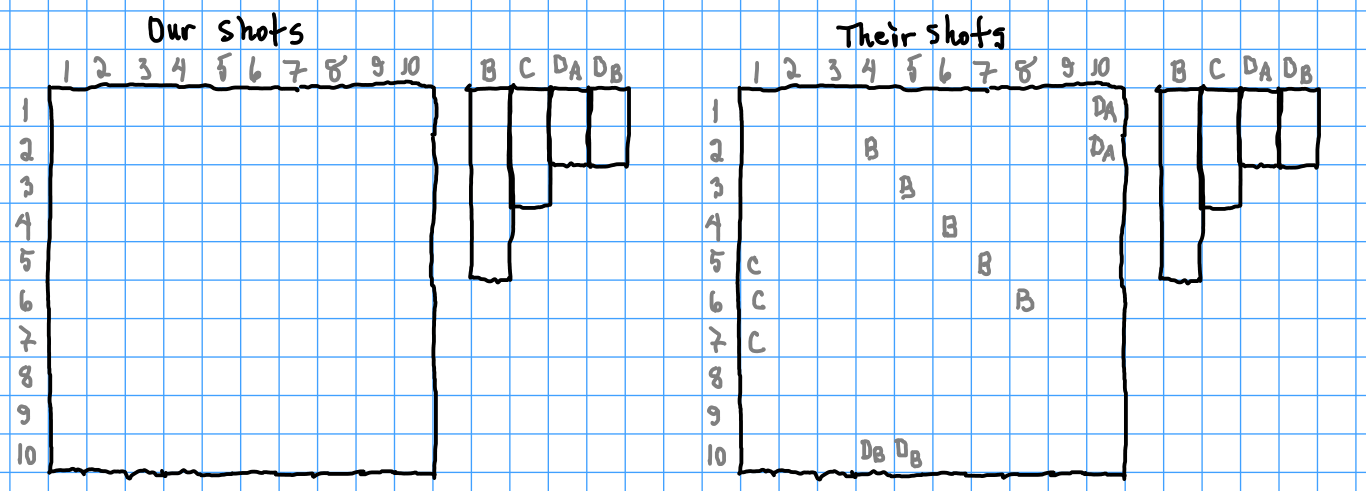I did not type the phrase "WHERE ARE YOUR SHIPS?" so no cheating (at least by the human) was done in this battle.

Since neither side knows the position of the enemy fleet, the battle begins by firing the salvos in a search pattern.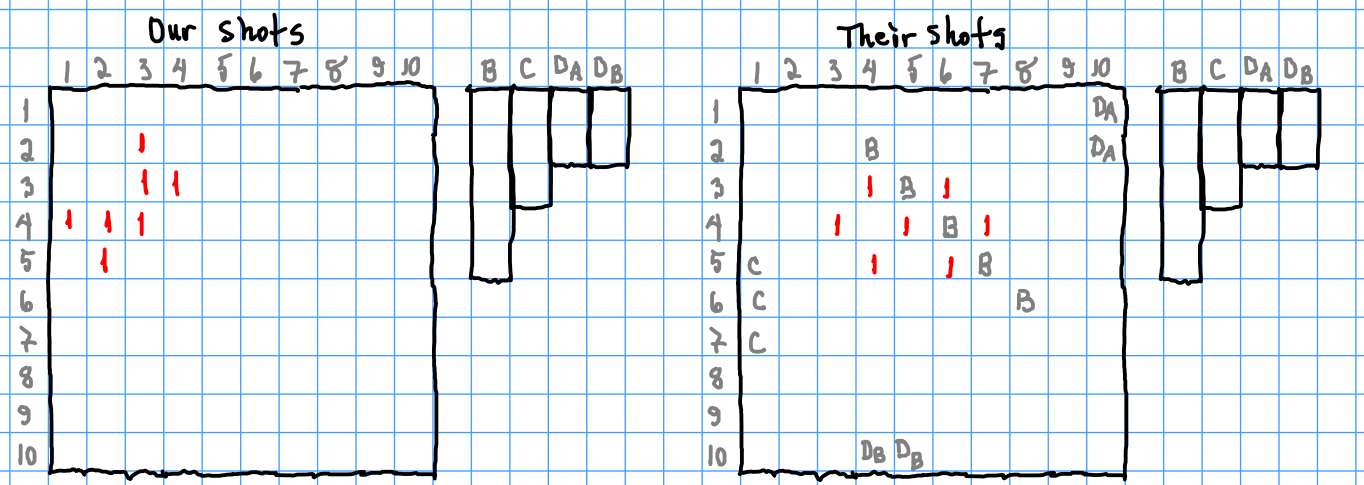It's worth observing that the figure 8 pattern used by the computer is generally designed for a game in which the ships are not allowed to be placed diagonally. On the other hand, in this game ships are allowed to be placed diagonally and the computer does not hesitate to do so with their own fleet. While both salvo's miss all ships, the computer's salvo is extremely unlucky because my battleship passes diagonally through it unscathed.

The next salvo is still in search mode.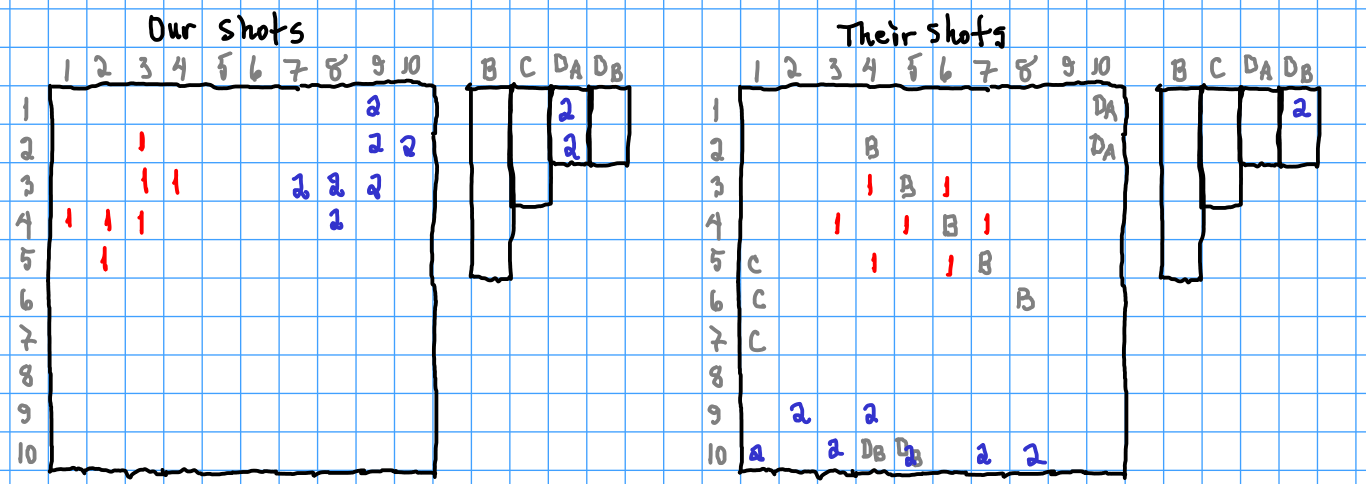I got lucky and sank a destroyer outright. The computer was also lucky, but not quite as lucky. Note that the hits for each round are recorded on the ship roster next to the grid so one can later search for matching patterns.

The computer sinks my destroyer on the third turn by interspersing a salvo with the previous salvo. I went back to searching and got lucky again.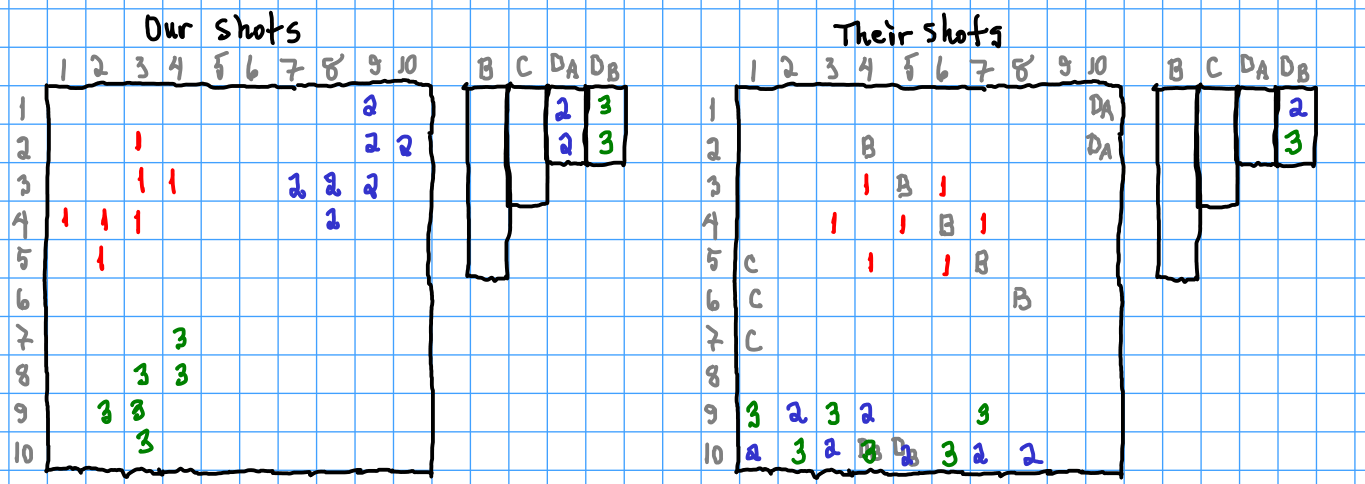On turn 4 the search for enemy ships continues since there are no ships that have been hit but not sunk. Weirdly the computer chose to search in the lower corner of the board near the other ships. The poorness of such a choice may have been random unluckiness that would even out in multiple battles.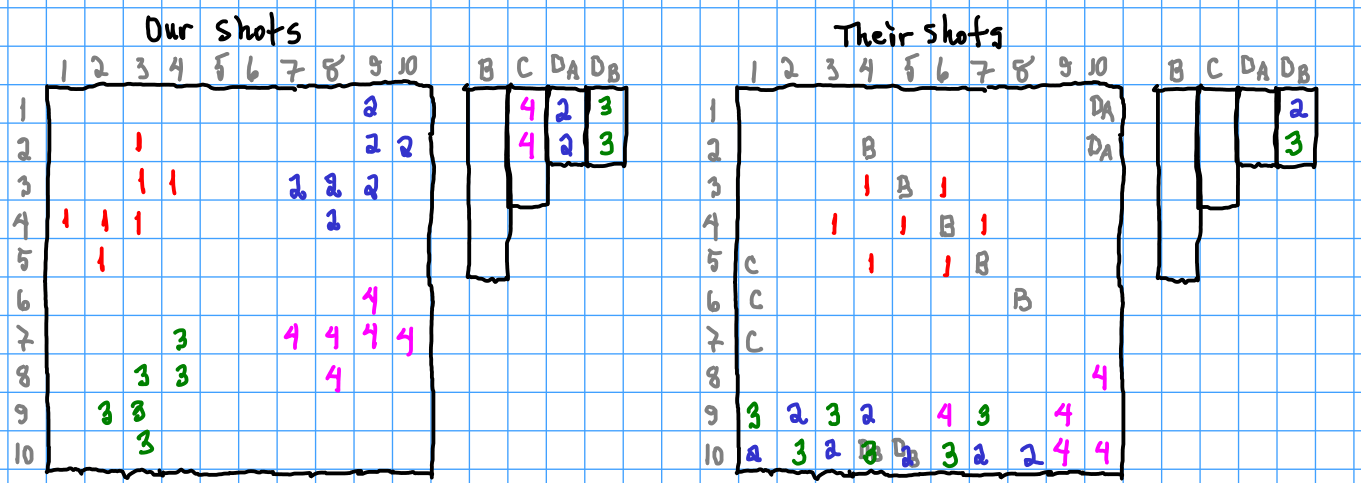Since I hit the cruiser twice in the previous round, I place the next salvo in a line where there are two 4's in a row. I also fire at (4,5) for good luck. The computer continues an unsuccessful search for my ships.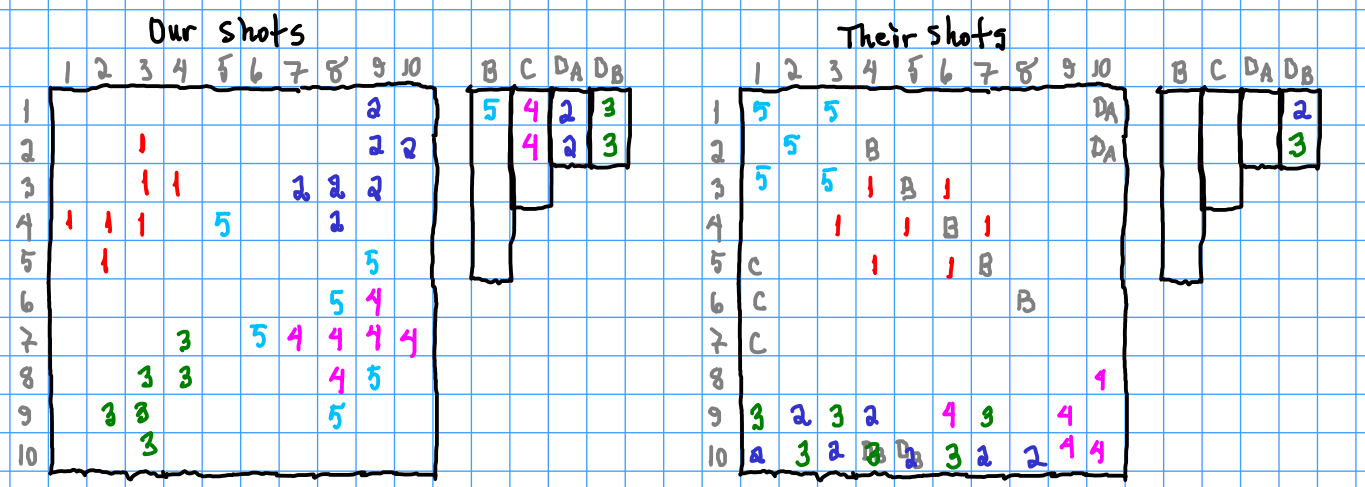Strangely, none of my missiles hit the cruiser, but I did by chance hit the enemy battleship. On turn 6 the computer again focuses on the lower right corner of the grid, which seems prone to failure and it fails. I look for the cruiser but this time along diagonals and succeed in sinking it.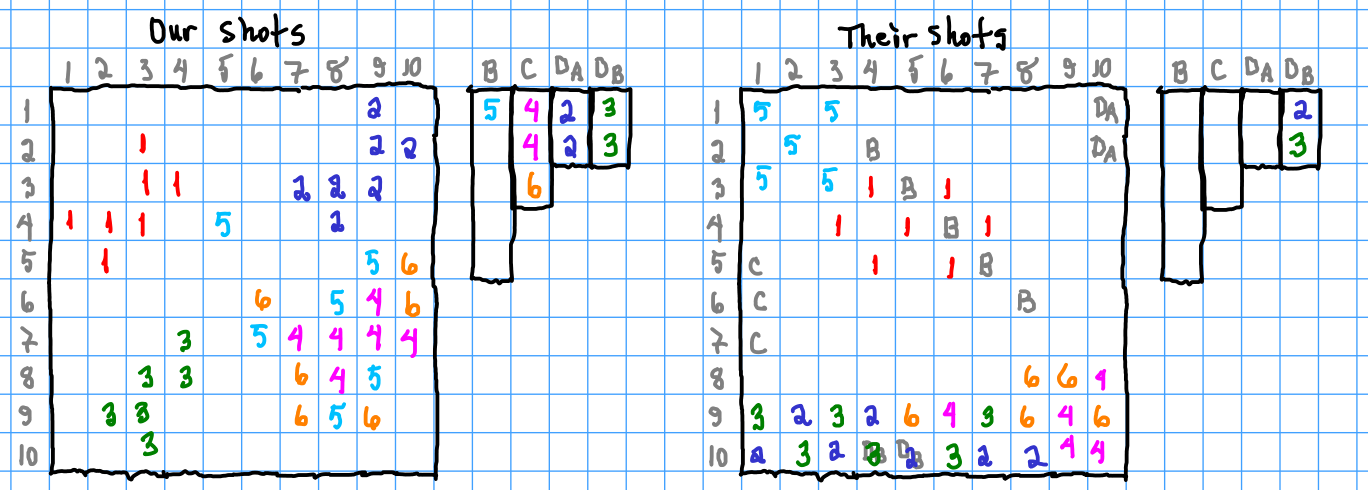On turn 7 the computer's search looked reasonable and hit my destroyer. For me there were four directions in which the enemy battleship could have passed though the shot at (4,5). In fact it could have been hit by almost any of the other missiles in the turn 5 salvo but I decided to focus on just one of them. Being ahead and hoping to end the game early I took a risk, lined up my shots vertically and won.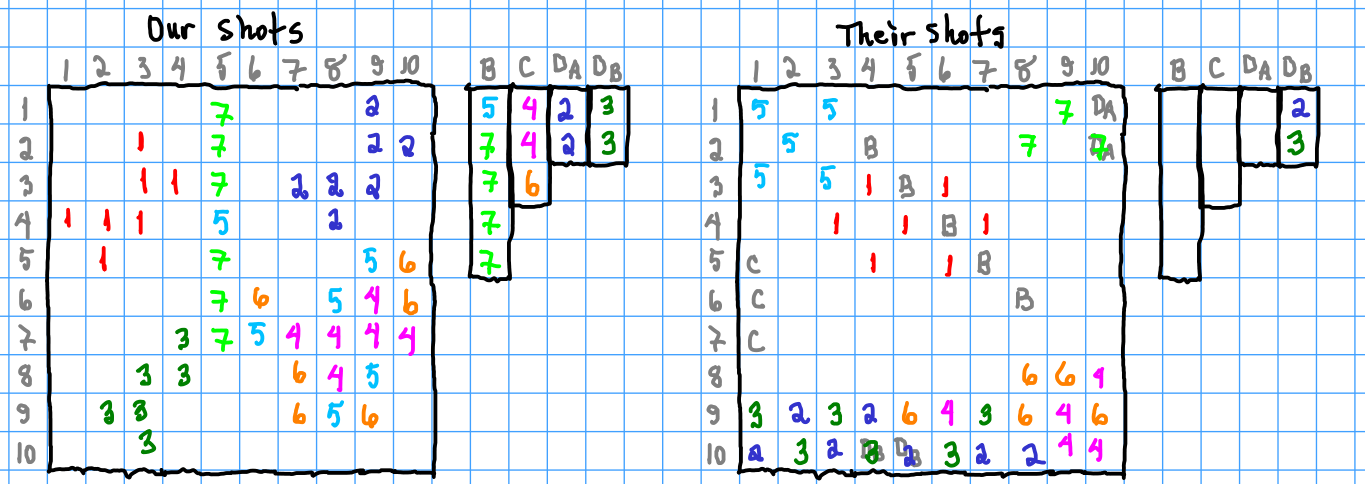The full transcript of the game is

Code: Select all

``````DO YOU WANT TO START? NO
ENTER COORDINATES FOR...
BATTLESHIP
? 2,4
? 3,5
? 4,6
? 5,7
? 6,8
CRUISER
? 5,1
? 6,1
? 7,1
DESTROYER<A>
? 1,10
? 2,10
DESTROYER<B>
? 10,4
? 10,5
DO YOU WANT TO SEE MY SHOTS? YES

TURN 1
I HAVE 7 SHOTS
3  4
4  5
3  6
4  3
5  4
5  6
4  7
YOU HAVE 7 SHOTS
? 2,3
? 3,3
? 3,4
? 4,1
? 4,2
? 4,3
? 5,2

TURN 2
I HAVE 7 SHOTS
9  2
10  3
9  4
10  1
10  5
10  7
10  8
YOU HAVE 7 SHOTS
? 1,9
? 2,9
? 2,10
? 3,7
? 3,8
? 3,9
? 4,8
YOU HIT MY DESTROYER<A>
YOU HIT MY DESTROYER<A>

TURN 3
I HAVE 6 SHOTS
9  3
10  2
10  4
10  6
9  7
9  1
YOU HAVE 6 SHOTS
? 7,4
? 8,3
? 8,4
? 9,2
? 9,3
? 10,3
YOU HIT MY DESTROYER<B>
YOU HIT MY DESTROYER<B>

TURN 4
I HAVE 5 SHOTS
9  6
10  9
8  10
9  9
10  10
YOU HAVE 6 SHOTS
? 6,9
? 7,7
? 7,8
? 7,9
? 7,10
? 8,8
YOU HIT MY CRUISER
YOU HIT MY CRUISER

TURN 5
I HAVE 5 SHOTS
1  1
2  2
1  3
3  1
3  3
YOU HAVE 6 SHOTS
? 4,5
? 5,9
? 6,8
? 7,6
? 8,9
? 9,8
YOU HIT MY BATTLESHIP

TURN 6
I HAVE 5 SHOTS
9  5
9  10
9  8
8  9
8  8
YOU HAVE 6 SHOTS
? 5,10
? 6,6
? 6,10
? 8,7
? 9,7
? 9,9
YOU HIT MY CRUISER

TURN 7
I HAVE 3 SHOTS
1  9
2  10
2  8
YOU HAVE 6 SHOTS
? 1,5
? 2,5
? 3,5
? 5,5
? 6,5
? 7,5
YOU HIT MY BATTLESHIP
YOU HIT MY BATTLESHIP
YOU HIT MY BATTLESHIP
YOU HIT MY BATTLESHIP

TURN 8
I HAVE 0 SHOTS
YOU HAVE WON

STOP at line 2900
``````
What would happen if the Basic program played against itself 333 times? Will the side that goes first first win significantly more often than the side which is defending? Does this have real-world implications?

I wonder whether my Fortran code plays any better.

What would happen if a program based on humanitarian principles tried its best not to sink the enemy ships? What if that program played against itself?
Last edited by ejolson on Thu Apr 28, 2022 5:08 pm, edited 1 time in total.

ejolson
Posts: 10725
Joined: Tue Mar 18, 2014 11:47 am

### Re: A Birthday Present for Fido

ejolson wrote:
Sat Apr 23, 2022 11:44 pm
What would happen if the Basic program played against itself 333 times? Will the side that goes first first win significantly more often than the side which is defending? Does this have real-world implications?
I've now have a somewhat debugged version of doggleship which runs two copies of the SALVO program from 101 Basic Computer Games in separate ptys so they can play each other. Thinking this would be a super good project to run on the Pi Zero cluster

viewtopic.php?p=1247739#p1247739

I copied the files to the head node and downloaded the latest console version of BBC Basic.

https://www.bbcbasic.co.uk/bbcbasic.html

Unfortunately, the result was

Code: Select all

``````\$ bbcbasic
Illegal instruction
``````
It would appear the official binary for BBC Basic was compiled for ARMv7 and the Zero has an ARMv6 processor. Just like the DOE is waiting for the Aurora exascale supercomputer, I'm still waiting for the Raspberry Pi 2 Zero.

Fortunately, BBC Basic is open source, so it's possible to build a working version.

Code: Select all

``````\$ git clone https://github.com/rtrussell/BBCSDL.git
\$ cd BBCSDL/console/raspi
``````
Then edit makefile so the changed lines look like

Code: Select all

``````#CXXFLAGS = -march=armv7-a -mthumb -munaligned-access -mfloat-abi=hard -c
CXXFLAGS = -munaligned-access -c
``````
Building the interpreter did not take long, even on a Zero.

To run the doggleship in parallel create five directories and copy the program, the Basic code and Fido's shell script with the cat into each one.

Code: Select all

``````\$ mkdir r1 r2 r3 r4 r5
\$ for i in r?; do cp bbcsalvo bbcsalvo.bbc doggleship \$i; done
``````
Then create a set of slurm submission files using the script

Code: Select all

``````#!/bin/bash
for i in r?
do
PWD=`pwd`
cat >\$i/doggleship.slm <<EOF
#!/bin/bash
#SBATCH -n 1
#SBATCH -c 1
#SBATCH -J doggle_\$i
#SBATCH -D \$PWD/\$i
time ./doggleship
EOF
done
``````
and launch the jobs with

Code: Select all

``````\$ for i in r?; do sbatch \$i/doggleship.slm; done
Submitted batch job 826
Submitted batch job 827
Submitted batch job 828
Submitted batch job 829
Submitted batch job 830
``````
The simulation is now running.

Since there is some latency when parsing the output from one copy of salvo and feeding it to the other, it does not run fast. I'm also expecting bugs or another race condition to prevent it from finishing. Note also I've scheduled 335 computer versus computer trials since 333 was not divisible by 5.

ejolson
Posts: 10725
Joined: Tue Mar 18, 2014 11:47 am

### Re: A Birthday Present for Fido

ejolson wrote:
Sun Apr 24, 2022 10:45 pm
The simulation is now running.

Since there is some latency when parsing the output from one copy of salvo and feeding it to the other, it does not run fast. I'm also expecting bugs or another race condition to prevent it from finishing. Note also I've scheduled 335 computer versus computer trials since 333 was not divisible by 5.
Amazingly the program finished without getting stuck in a race anywhere. After a small cleanup so it accurately determined who won, I obtained out of 335 runs that the fleet which fires the first salvo wins the battle 63 percent of the time.

The average length of the game was 17.7 turns, which seems high to me and may reflect non-optimal play by the Basic program. I wonder whether the typo in line 3970 in the microcomputer edition leads to better or worse strategy. Stay tuned for the typo versus original program playoff.

For reference the source code for the current doggleship program is

Code: Select all

``````/*  doggleship.go--computer versus computer salvo
Written 2022 by Eric Olson

Version 1 run SALVO from 101 Basic Computer Games */

package main

import (
"fmt"; "os"; "syscall"; "unsafe"; "C"
"os/exec"; "golang.org/x/term"; "bufio"
"time"; "strings"; "strconv" )

type (
stspec int
cospec struct {
i,j int
}
btspec struct {
s string
p []cospec
}
gmspec struct {
b []btspec
sr []string
pr *os.Process
fd *os.File
first bool
cmd,log string
}
)

const ( running stspec=iota; lost; won; twait=time.Duration(1) )

func spawn(cl string)(*os.File,*os.Process) {
fmaster,err:=os.OpenFile("/dev/ptmx",os.O_RDWR,0)
if err!=nil {
fmt.Printf("Error opening /dev/ptms for read and write!\n")
os.Exit(1)
}
var pn string
{
var n C.uint
syscall.Syscall(syscall.SYS_IOCTL,fmaster.Fd(),
syscall.TIOCGPTN,uintptr(unsafe.Pointer(&n)))
pn=fmt.Sprintf("/dev/pts/%d",n)
n=0
syscall.Syscall(syscall.SYS_IOCTL,fmaster.Fd(),
syscall.TIOCSPTLCK,uintptr(unsafe.Pointer(&n)))
}
fslave,err:=os.OpenFile(pn,os.O_RDWR,0)
term.MakeRaw(int(fslave.Fd()))
if err!=nil {
fmt.Printf("Error opening %s for read and write\n%v!\n",pn,err)
os.Exit(1)
}
cmd:=exec.Command(cl)
cmd.Stdin=fslave; cmd.Stdout=fslave; cmd.Stderr=fslave
cmd.Start()
return fmaster,cmd.Process
}

func monitor(sr *[]string,fp *os.File,log string) {
logio,err:=os.Create(log)
if err!=nil {
fmt.Printf("Error opening %s for write!\n",log)
os.Exit(1)
}
defer logio.Close()
for {
var b byte
if err!=nil { return }
var i,j int
for i,j=0,0;j<n;j++ {
if b[j]=='\n' {
i=j+1
} else if b[j]=='\r' {
(*sr)[len(*sr)-1]+=string(b[i:j])
fmt.Fprintf(logio,"%s\n",b[i:j])
*sr=append(*sr,"")
i=j+1
}
}
if i<j {
(*sr)[len(*sr)-1]+=string(b[i:j])
fmt.Fprintf(logio,"%s",b[i:j])
}
}
}

func istail(a,b string)bool {
if len(a)<len(b) { return false }
if a[len(a)-len(b):]==b { return true }
return false
}

if len(a)<len(b) { return false }
if a[0:len(b)]==b { return true }
return false
}

func mkgame(first bool)gmspec {
var game gmspec
game.b=[]btspec{
btspec{"BATTLESHIP",make([]cospec,0,5)},
btspec{"CRUISER",make([]cospec,0,5)},
btspec{"DESTROYER<A>",make([]cospec,0,5)},
btspec{"DESTROYER<B>",make([]cospec,0,5)},
}
game.sr=make([]string,1,512)
game.first=first
return game
}

func prboats(b []btspec){
fmt.Printf("\n")
for i:=0;i<len(b);i++ {
fmt.Printf("|%s\n",b[i].s)
for j:=0;j<len(b[i].p);j++ {
fmt.Printf("| %d  %d\n",b[i].p[j].i,b[i].p[j].j)
}
}
}

func (game *gmspec)findtail(s string)bool {
t:=twait
for p:=len(game.sr)-1;!istail(game.sr[p],s);p=len(game.sr)-1 {
time.Sleep(t*time.Millisecond)
if game.sr[p]==">" { return false }
if t<128 { t*=2 }
}
return true
}

func (game *gmspec)nextprompt(p int)int {
t:=twait
for {
game.findtail("? ")
r:=len(game.sr)
if r>p { return r }
time.Sleep(t*time.Millisecond)
if t<128 { t*=2 }
}
}

func (game *gmspec)bbcboot(cmd,log string) {
getname:=func(s string)int {
for j:=0;j<len(game.b);j++ {
if s==game.b[j].s {
return j
}
}
return -1
}
game.cmd=cmd; game.log=log
game.fd,game.pr=spawn(cmd)
go monitor(&game.sr,game.fd,log)
game.findtail("DO YOU WANT TO START? ")
p:=len(game.sr)
game.nextprompt(p)
j:=-1
for i:=p;i<len(game.sr);i++ {
r:=getname(game.sr[i])
if r>=0 { j=r
} else if j>=0{
sxy:=strings.Fields(game.sr[i])
if len(sxy)==2 {
x,err:=strconv.Atoi(sxy)
if err!=nil { continue }
y,err:=strconv.Atoi(sxy)
if err!=nil { continue }
game.b[j].p=append(game.b[j].p,cospec{x,y})
}
}
}
if game.first {
fmt.Fprintf(game.fd,"NO\n")
} else {
fmt.Fprintf(game.fd,"YES\n")
}
}

func (game *gmspec)bbcplace(b []btspec){
p:=len(game.sr)-1
for i:=0;i<len(b);i++ {
for j:=0;j<len(b[i].p);j++ {
p=game.nextprompt(p)
fmt.Fprintf(game.fd,"%d,%d\n",
b[i].p[j].i,b[i].p[j].j)
}
}
game.findtail("DO YOU WANT TO SEE MY SHOTS? ")
p=len(game.sr)
fmt.Fprintf(game.fd,"YES\n")
game.nextprompt(p)
}

func (game *gmspec)bbcoutgoing(r []cospec){
p:=len(game.sr)-1
for i:=0;i<len(r);i++ {
p=game.nextprompt(p)
fmt.Fprintf(game.fd,"%d,%d\n",r[i].i,r[i].j)
}
game.nextprompt(p)
}

func (game *gmspec)bbcincoming()([]cospec,stspec) {
r:=make([]cospec,0,7)
var p int
for p=len(game.sr)-1;p>=0;p-- {
return r,won
} else if ishead(game.sr[p],"YOU HAVE WON"){
return r,lost
} else if ishead(game.sr[p],"I HAVE "){
break
}
}
sn:=strings.Fields(game.sr[p])
if len(sn)!=4 {
fmt.Printf("%s:Couldn't parse number of shots from '%s'\n",
game.log,game.sr[p])
os.Exit(1)
}
n,err:=strconv.Atoi(sn)
if err!=nil {
fmt.Printf("%s:Couldn't convert '%s' to number of shots\n",
game.log,sn)
os.Exit(1)
}
p++; n+=p
for i:=p;i<n;i++ {
sxy:=strings.Fields(game.sr[i])
if len(sxy)==2 {
x,err:=strconv.Atoi(sxy)
if err!=nil { continue }
y,err:=strconv.Atoi(sxy)
if err!=nil { continue }
r=append(r,cospec{x,y})
}
}
return r,running
}

func dotrial(trial int){
game1:=mkgame(true)
game2:=mkgame(false)
game1.bbcboot("./bbcsalvo",fmt.Sprintf("s%04d_1.txt",trial))
game2.bbcboot("./bbcsalvo",fmt.Sprintf("s%04d_2.txt",trial))
game1.bbcplace(game2.b)
game2.bbcplace(game1.b)
for turn:=1;;turn++ {
r1,status:=game1.bbcincoming()
if status==lost {
fmt.Printf("%7d %7d %7d\n",trial,2,turn)
break
} else if status==won {
fmt.Printf("%7d %7d %7d\n",trial,1,turn)
break
} else {
game2.bbcoutgoing(r1)
}
r2,status:=game2.bbcincoming()
if status==lost {
fmt.Printf("%7d %7d %7d\n",trial,1,turn)
break
} else if status==won {
fmt.Printf("%7d %7d %7d\n",trial,2,turn)
break
} else {
game1.bbcoutgoing(r2)
}
}
game1.fd.Close()
game2.fd.Close()
if game1.pr!=nil { game1.pr.Signal(syscall.SIGINT) }
if game2.pr!=nil { game2.pr.Signal(syscall.SIGINT) }
}

func main() {
fmt.Printf("doggleship--computer versus computer salvo\n"+
"Written 2022 by Eric Olson\n\n")
fmt.Printf("%7s %7s %7s\n","Trial","Winner","Turns")
for trial:=1;trial<=67;trial++ {
dotrial(trial)
}
os.Exit(0)
}
``````
Note that it doesn't properly clean up if interrupted, so proceed with caution.

I wonder whether it would be easier to create a driver for the Fortran program or adapt the Fortran code so it presents the same prompts and output as the Basic.
Last edited by ejolson on Thu Apr 28, 2022 5:15 am, edited 2 times in total.

ejolson
Posts: 10725
Joined: Tue Mar 18, 2014 11:47 am

### Re: A Birthday Present for Fido

ejolson wrote:
Mon Apr 25, 2022 3:45 am
I wonder whether it would be easier to create a driver for the Fortran program or adapt the Fortran code so it presents the same prompts and output as the Basic.
Today the weather stopped oscillating between Winter and Summer. As there was no snow nor wind providing a disincentive, I spent some time outside watching the quail hop about until the presence of the dog developer became known and they fluttered away.

Having nothing else to do, I decided to ask Fido about updating the Fortran battleship program.
jahboater wrote:
Sun Apr 17, 2022 9:12 am
After showing off my newly acquired book "Modern Fortran Explained" by Metcalf, Reid and Cohen, the super pet pointed out that the SuperPET runs only valuable legacy code.microFortran.png (11.41 KiB) Viewed 2451 times
Since it is a birthday present for Fido, I made a mental note to look for a different book after returning home.

The next few hours passed pleasantly enough without pheasants and only a bit of barking. After returning home I discovered the shelf was empty. Then I remembered the old Fortran books had been donated to a charitable cause--the doghouse library--many years ago.

Shortly after I sent in the digital library loan request, I received a scanned book through FidoNET.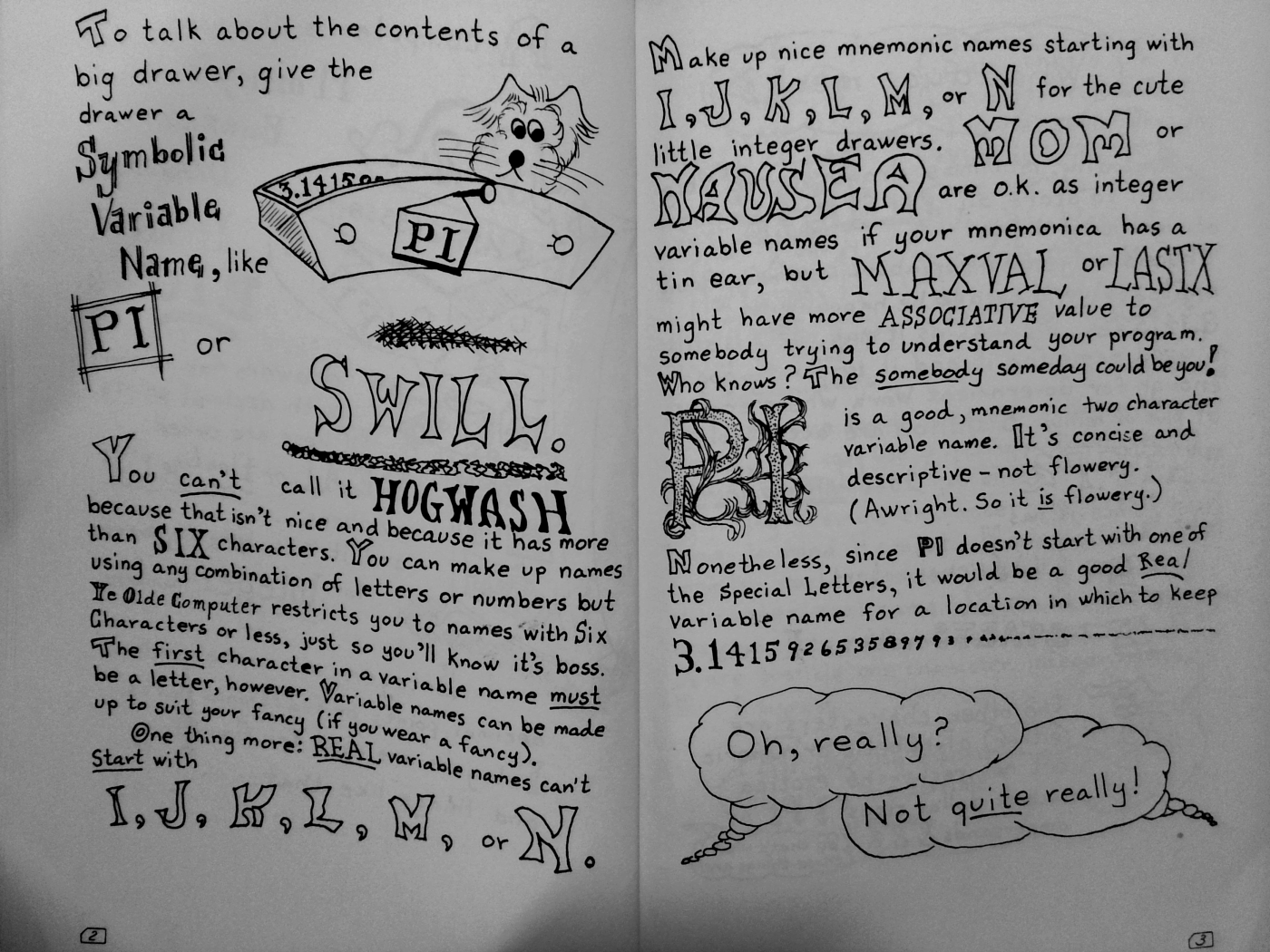https://archive.org/details/9780262610261

Upon glancing at Dr Kaufman's "A Fortran Coloring Book" I suddenly remembered why the Fortran program did not have the same user interface as the Basic.

It further occurred to me it never would.
Last edited by ejolson on Thu Apr 28, 2022 5:44 am, edited 1 time in total.

ejolson
Posts: 10725
Joined: Tue Mar 18, 2014 11:47 am

### Re: A Birthday Present for Fido

ejolson wrote:
Mon Apr 25, 2022 10:15 pm
the SuperPET runs only valuable legacy code.
Woohoo! In a triumph of birthday-present madness my code (originally written in Microsoft Fortran) is running on the SuperPET emulator hosted by a Raspberry Pi 4B and accessed through remote desktop.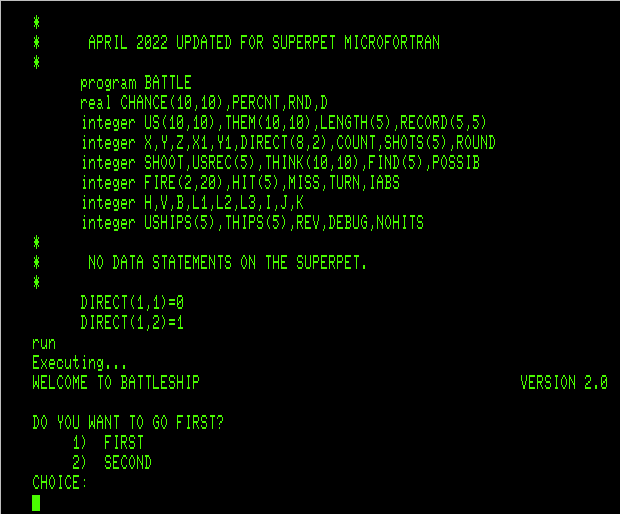Given the interpretive nature of the Waterloo languages, it does not run fast. After I manage to finish one game by hand, I'll post the source along with the results of who won.

Would it run faster with IBM Fortran H on the Herculus emulator?
Last edited by ejolson on Thu Apr 28, 2022 12:29 am, edited 1 time in total.

ejolson
Posts: 10725
Joined: Tue Mar 18, 2014 11:47 am

### Re: A Birthday Present for Fido

ejolson wrote:
Tue Apr 26, 2022 8:56 pm
Given the interpretive nature of the Waterloo languages, it does not run fast. After I manage to finish one game by hand, I'll post the source along with the results of who won.
Even with warp mode enabled the SuperPET ran a bit slow. Maybe that's why the dog developer was not able to catch those quail in the park. Even so, I managed to finish a game. Since the game-play was notably different than the Basic code, I'll include another tedious turn by turn account with commentary.

The SuperPET went first and was quite lucky. On turn 1 it managed to hit my battleship twice and the cruiser once. My return salvo was a miss.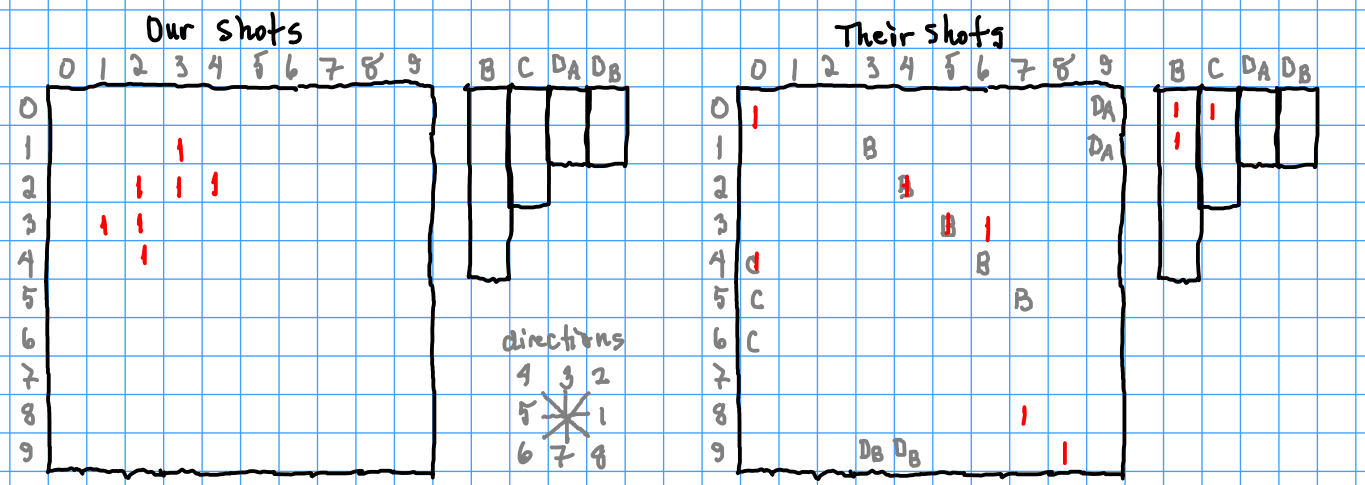On turn 2 the SuperPET sank my battleship. The Fortran algorithm counts all possible places the ships could be and then launches a salvo to cover the most likely positions as best as possible. Unlike some real-world examples, the missiles actually hit the locations targeted.

Since the results from the first salvo narrowed the locations for the battleship to nine possibilities, the second salvo was chosen in a way guaranteed to sink my battleship in three of the nine possibilities and at least hit it in eight of them. There was only one possibility in the lower right corner where the battleship could have escaped being hit.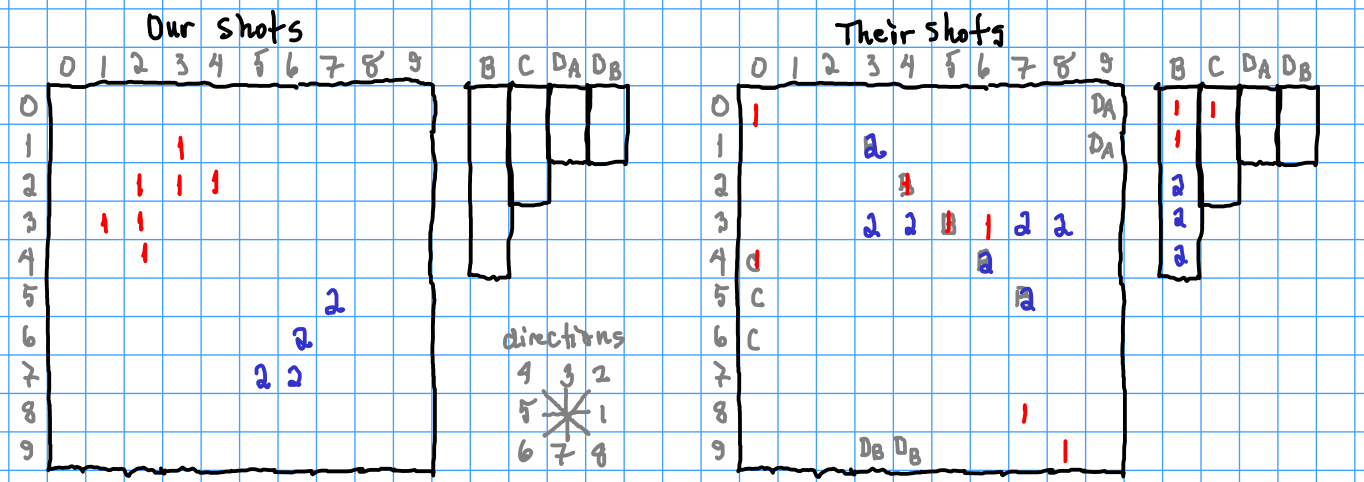On turn 3 the number of available missiles I had for the counter strike was reduced to four. An early loss like this is why the side which goes first has an advantage.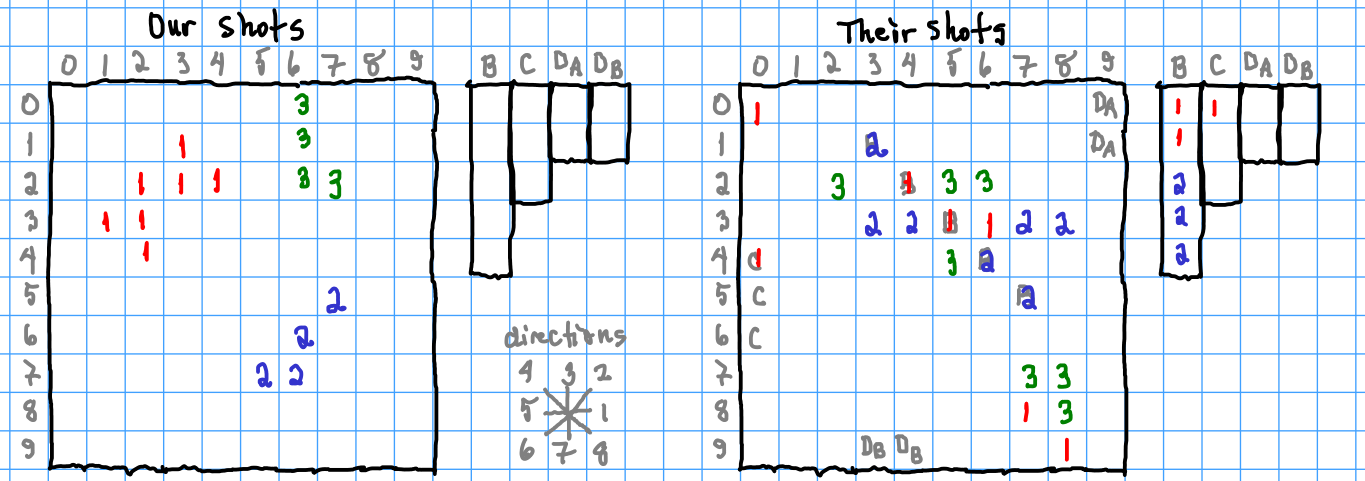On turn 4 the SuperPET scores another hit on my cruiser. That was expected as the algorithm automatically focuses on ships whose location is better known by having been hit before.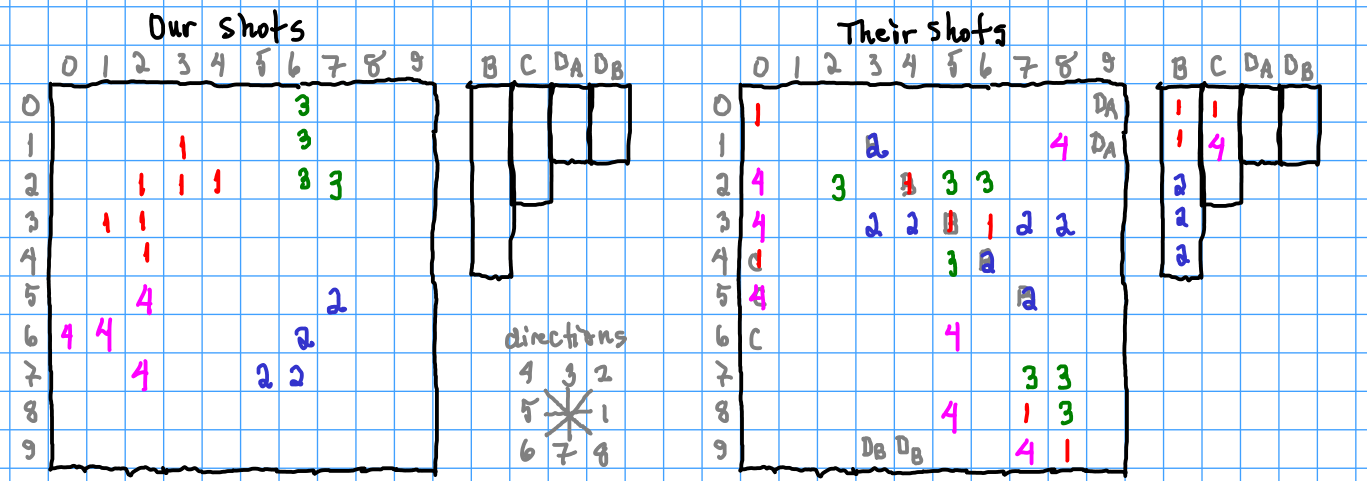Not surprisingly my cruiser was sunk in the next round as there were very few possible locations where a ship of length three could be hiding that had already been hit in the first and fourth rounds. This reduced my counter attack to only two missiles, but I got lucky and hit the battleship twice.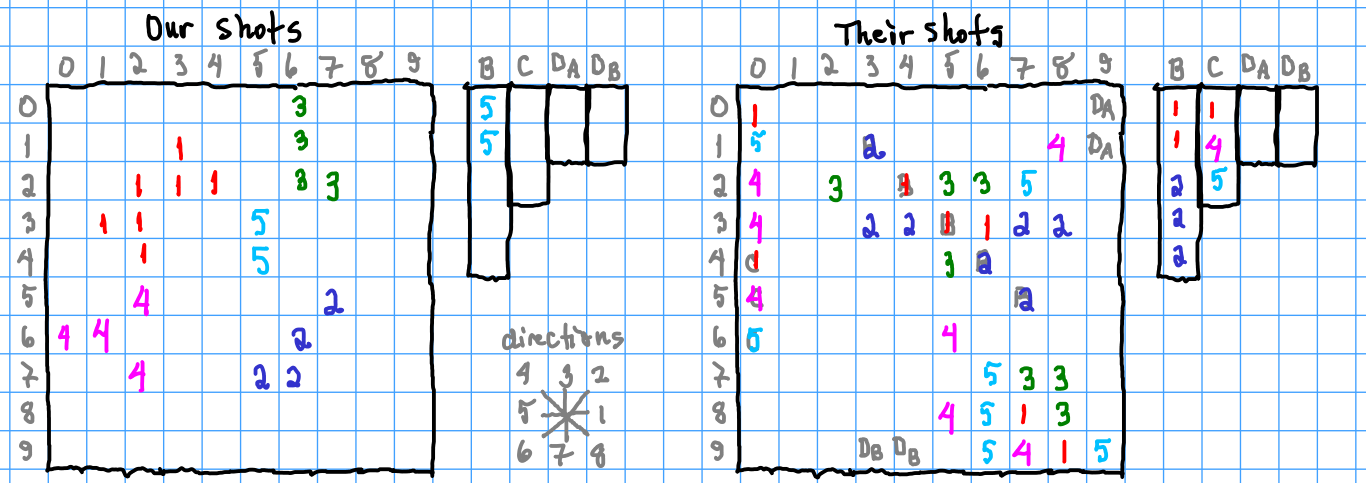There were three places the battleship could be. I chose positions that were as likely as possible but hit only once.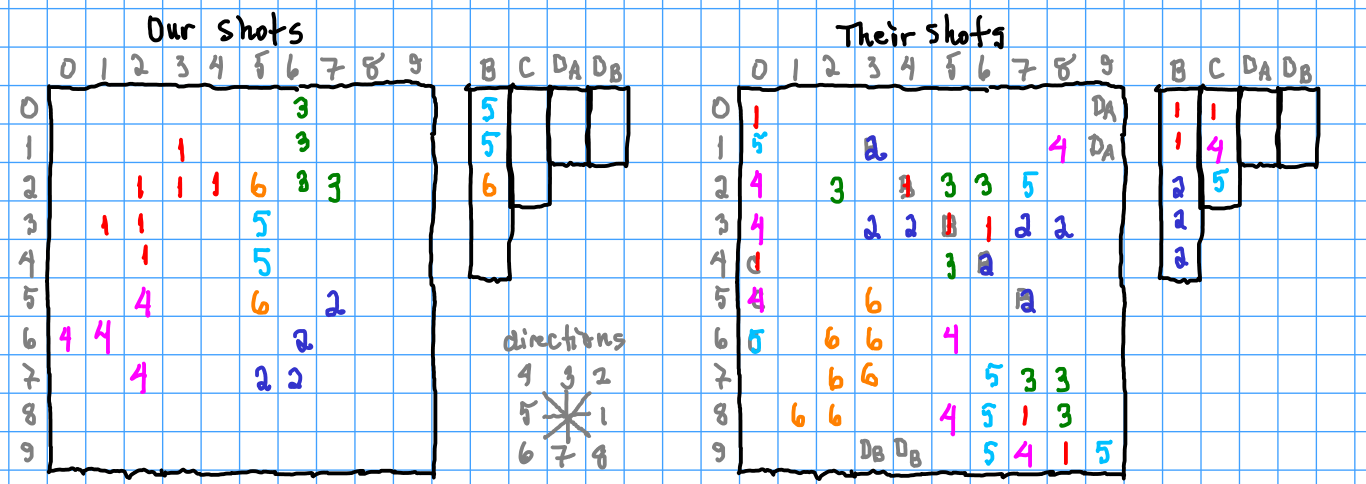I was able to sink the battleship on turn 7 and felt I might even have a chance to win since the SuperPET had not hit either of my destroyers.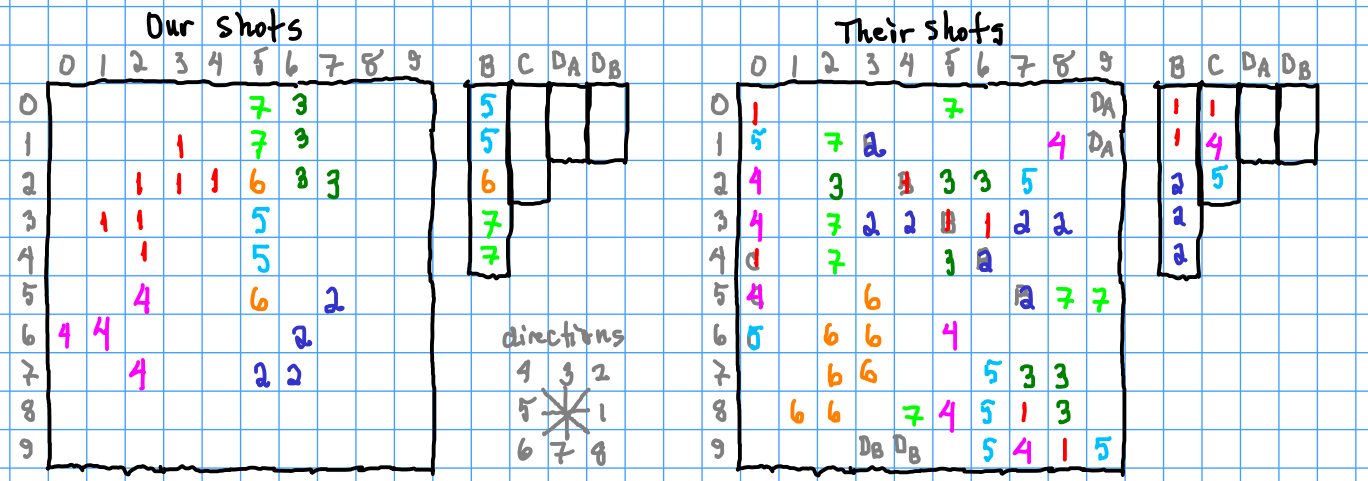Since the SuperPET has twice as many missiles in each salvo it is able to search faster. Otherwise, nothing happened.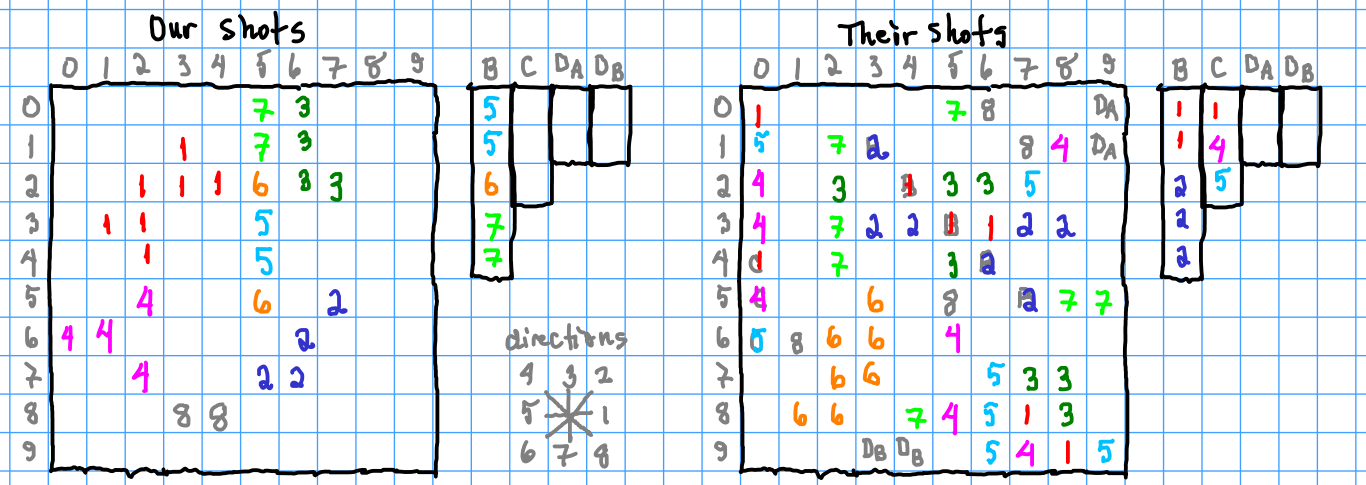On turn 9 my luck ran out and one destroyer was hit. Worse luck was my second destroyer was close to one of the strikes in that salvo and would likely receive a collateral hit when the SuperPET attempts to sink the first destroyer.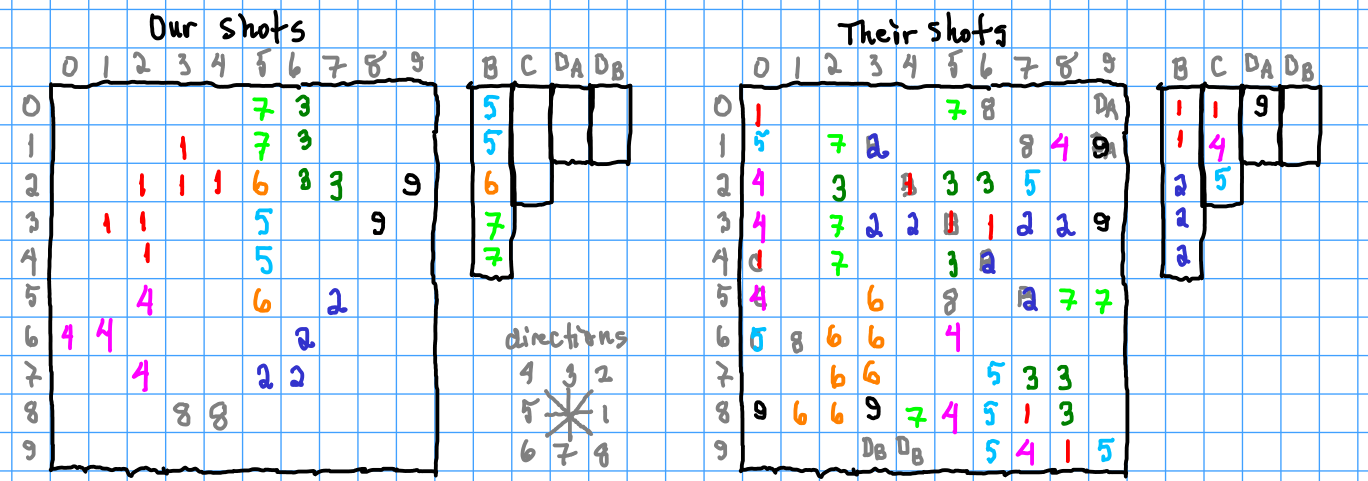On turn 10 that's exactly what happened. The second destroyer was hit while the SuperPET was searching for the first.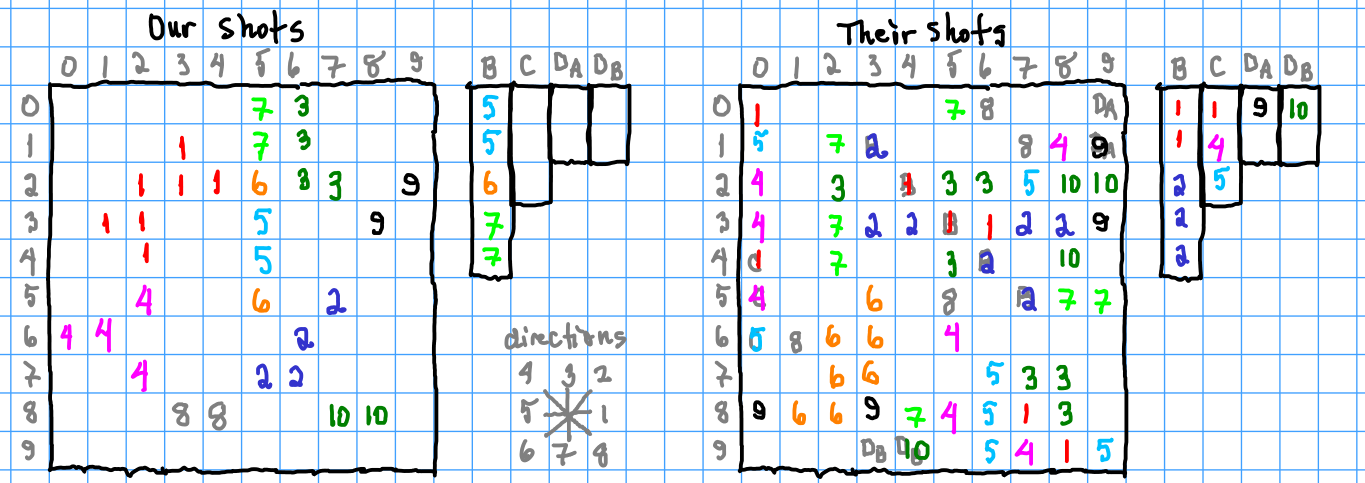By turn 11 I'm down to only one missile. As no friendly country has donated any additional weapons to my defense (even though the SuperPET went first), the end seems near.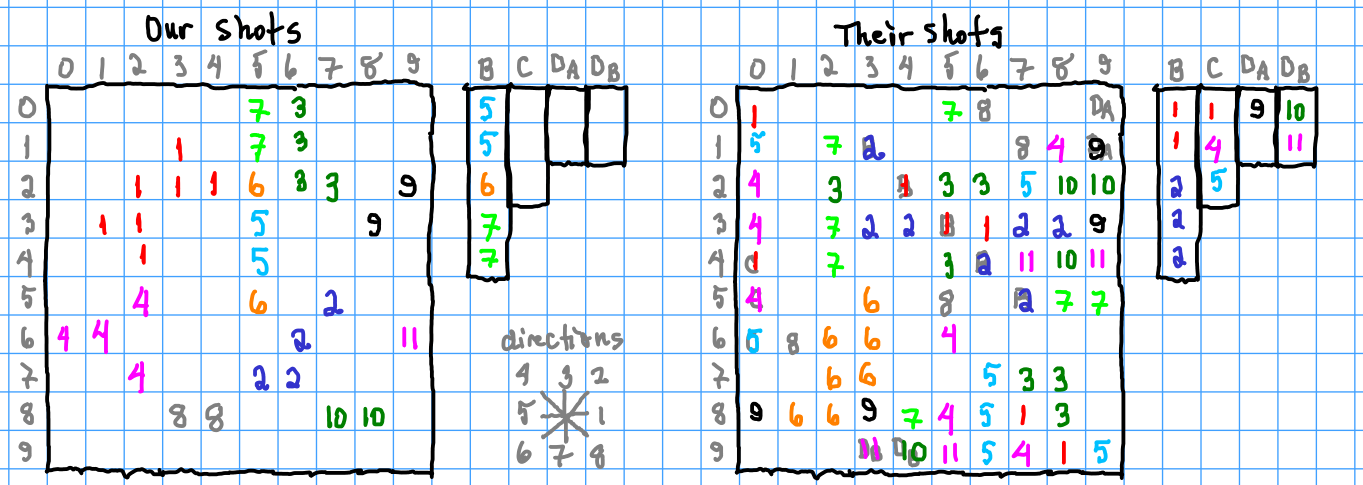The game ends on the next turn with a victory in which the enemy lost only the battleship. Even so, I had the last laugh by shutting down the emulated SuperPET with alt-Q and taking the real super pet to the park.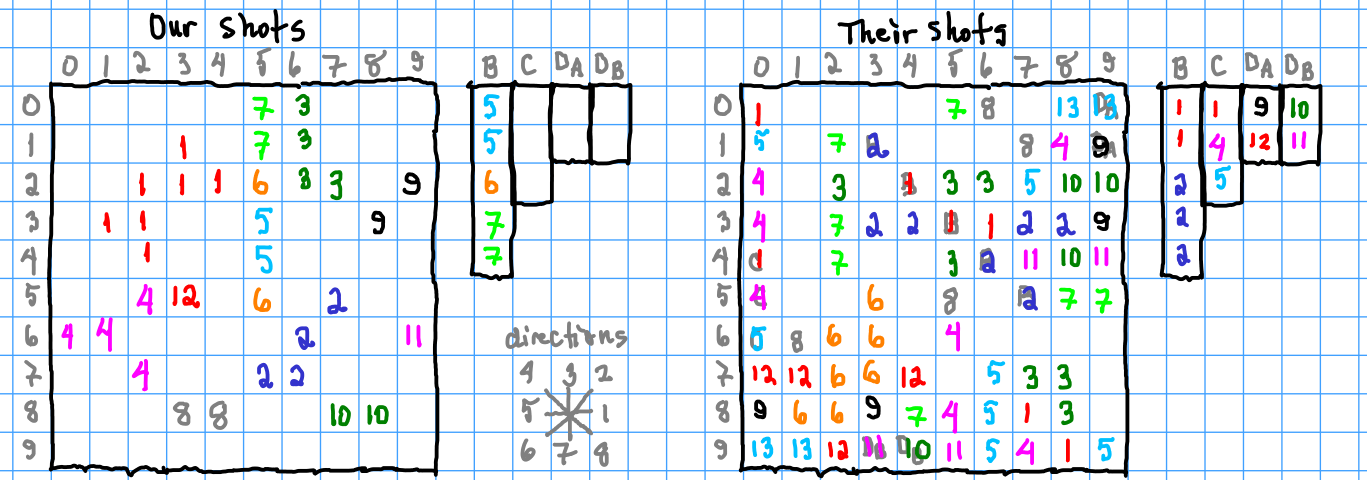The source code modified for Waterloo microFortran is

Code: Select all

``````*
*      BATTLESHIP  V2.1
*      BY ERIC OLSON
*      JUNE 6, 1983
*
*      APRIL 2022 UPDATED FOR SUPERPET MICROFORTRAN
*
PROGRAM BATTLE
REAL CHANCE(10,10),PERCNT,RND,D
INTEGER US(10,10),THEM(10,10),LENGTH(5),RECORD(5,5)
INTEGER X,Y,Z,X1,Y1,DIRECT(8,2),COUNT,SHOTS(5),ROUND
INTEGER SHOOT,USREC(5),THINK(10,10),FIND(5),POSSIB
INTEGER FIRE(2,20),HIT(5),MISS,TURN,IABS
INTEGER H,V,B,L1,L2,L3,I,J,K
INTEGER USHIPS(5),THIPS(5),REV,DEBUG,NOHITS
*
*      NO DATA STATEMENTS ON THE SUPERPET.
*
DIRECT(1,1)=0
DIRECT(1,2)=1
DIRECT(2,1)=-1
DIRECT(2,2)=1
DIRECT(3,1)=-1
DIRECT(3,2)=0
DIRECT(4,1)=-1
DIRECT(4,2)=-1
DIRECT(5,1)=0
DIRECT(5,2)=-1
DIRECT(6,1)=1
DIRECT(6,2)=-1
DIRECT(7,1)=1
DIRECT(7,2)=0
DIRECT(8,1)=1
DIRECT(8,2)=1
LENGTH(1)=5
LENGTH(2)=3
LENGTH(3)=2
LENGTH(4)=2
LENGTH(5)=0
SHOTS(1)=3
SHOTS(2)=2
SHOTS(3)=1
SHOTS(4)=1
SHOTS(5)=0
DO 91 X=1,10
DO 91 Y=1,10
US(X,Y)=0
91 THEM(X,Y)=0
DO 92 X=1,5
DO 92 Y=1,5
92 RECORD(X,Y)=0
*
*      SET RUN OPTIONS.
*
NOHITS=1
DEBUG=0
ROUND=0
*
*      DISPLAY WELCOME MESSAGE.
*
WRITE(6,1000)
*
*      SET UP SHIPS.
*
DO 10 I=1,5
USREC(I)=LENGTH(I)
USHIPS(I)=1
THIPS(I)=1
IF(LENGTH(I).EQ.0) USHIPS(I)=0
IF(LENGTH(I).EQ.0) THIPS(I)=0
10 CONTINUE
*
*      COMPUTER POSITION BOATS.
*
DO 50 I=1,5
IF(LENGTH(I).EQ.0) GOTO 50
45 X=IFIX(RND(0.0)*10.0)+1
Y=IFIX(RND(0.0)*10.0)+1
Z=IFIX(RND(0.0)*4.0)+1
X1=X
Y1=Y
COUNT=LENGTH(I)
*
*      FIND A LOCTION AND TEST TO SEE IF IT FITS.
*
47 IF(US(X1,Y1).NE.0) GOTO 45
X1=X1+DIRECT(Z,1)
Y1=Y1+DIRECT(Z,2)
COUNT=COUNT-1
IF(COUNT.LE.0) GOTO 9301
IF(Y1.GT.10.OR.X1.GT.10.OR.Y1.LT.1.OR.X1.LT.1) GOTO 45
GOTO 47
9301 X1=X
Y1=Y
COUNT=LENGTH(I)
*
*      PUT THE BOAT THERE.
*
48 US(X1,Y1)=-I
X1=X1+DIRECT(Z,1)
Y1=Y1+DIRECT(Z,2)
COUNT=COUNT-1
IF(COUNT.GT.0) GOTO 48
50 CONTINUE
*
*      WHO GOES FIRST?
*
51 WRITE(6,1200)
REV=0
IF(I.NE.0) GOTO 101
CALL BOARD(US)
GOTO 51
101 IF(I.EQ.2) REV=1
IF(I.EQ.1.OR.I.EQ.2) GOTO 111
110 WRITE(6,1220)
GOTO 100
111 CONTINUE
*
*      HUMAN POSITION BOATS.
*
DO 90 I=1,5
IF(LENGTH(I).EQ.0) GOTO 90
WRITE(6,1020) LENGTH(I)
*
*      ENTER STARTING LOCATION.
*
IF(X.GE.0.AND.X.LT.10.AND.Y.GE.0.AND.Y.LT.10) GOTO 65
60 WRITE(6,1030)
GOTO 55
65 X1=X+1
Y1=Y+1
COUNT=LENGTH(I)
WRITE(6,1040)
*
*      ENTER DIRECTION.
*
IF(Z.GT.0.AND.Z.LE.8) GOTO 68
67 WRITE(6,1050)
GOTO 66
*
*      TEST TO SEE IF IT FITS.
*
68 IF(THEM(X1,Y1).NE.0) GOTO 69
X1=X1+DIRECT(Z,1)
Y1=Y1+DIRECT(Z,2)
COUNT=COUNT-1
IF(COUNT.LE.0) GOTO 9302
IF(Y1.GT.10.OR.X1.GT.10.OR.Y1.LT.1.OR.X1.LT.1) GOTO 69
GOTO 68
9302 X1=X+1
Y1=Y+1
COUNT=LENGTH(I)
GOTO 70
*
*      IT DOESN'T FIT!
*
69 WRITE(6,1080)
GOTO 60
*
*      PUT THE BOAT THERE.
*
70 THEM(X1,Y1)=-I
X1=X1+DIRECT(Z,1)
Y1=Y1+DIRECT(Z,2)
COUNT=COUNT-1
IF(COUNT.GT.0) GOTO 70
90 CONTINUE
*
*      COUNT SHOTS AND HAVE THE HUMAN ENTER THEM.
*
IF(REV.EQ.1) GOTO 3000
2000 CONTINUE
2 MISS=0
DO 14 L1=1,5
14 HIT(L1)=0
IF(REV.EQ.0) ROUND=ROUND+1
I=SHOOT(THIPS,SHOTS)
WRITE(6,1230) ROUND,I
IF(I.GT.0) GOTO 124
WRITE(6,1310)
STOP
124 DO 160 J=1,I
WRITE(6,1240) J
IF(X.GE.0.AND.Y.GE.0.AND.X.LE.9.AND.Y.LE.9) GOTO 130
127 WRITE(6,1030)
GOTO 125
130 IF(US(X+1,Y+1).EQ.0) GOTO 140
K=IABS(US(X+1,Y+1))
HIT(K)=HIT(K)+1
USREC(K)=USREC(K)-1
US(X+1,Y+1)=0
IF(USREC(K).GT.0) GOTO 160
USHIPS(K)=0
HIT(K)=-IABS(HIT(K))
GOTO 160
140 MISS=MISS+1
160 CONTINUE
*
*      TELL HIM WHAT HE HIT!
*
WRITE(6,1520) MISS
DO 161 L1=1,5
IF(HIT(L1).EQ.0) GOTO 161
L2=IABS(HIT(L1))
WRITE(6,1500) L1,L2
IF(HIT(L1).LT.0) WRITE(6,1510)
161 CONTINUE
GOTO 3000
*
*      COMPUTER TAKES HIS TURN.
*
3000 CONTINUE
3 MISS=0
DO 162 L1=1,5
162 HIT(L1)=0
IF(REV.EQ.1) ROUND=ROUND+1
I=SHOOT(USHIPS,SHOTS)
WRITE(6,1280) ROUND,I
IF(I.GT.0) GOTO 163
WRITE(6,1320)
STOP
*
*      RANDOM IF FIRST OR SECOND ROUND AND NOTHING HIT.
*
163 IF(NOHITS.EQ.0) GOTO 199
IF(ROUND.GT.2) GOTO 199
DO 198 X=1,10
DO 198 Y=1,10
198 CHANCE(X,Y)=1E0
GOTO 1811
*
*      INITIALIZE THINK.
*
199 DO 200 J=1,10
DO 200 K=1,10
200 CHANCE(J,K)=0E0
*
*      EXAMINE ALL POSSIBLE SHIPS.
*
DO 4000 B=1,5
IF(THIPS(B).EQ.0) GOTO 4000
POSSIB=0
DO 1812 J=1,10
DO 1812 K=1,10
1812 THINK(J,K)=0
*
*      EXAMINE ALL PLACES.
*
DO 4002 H=1,10
DO 4002 V=1,10
*
*      POSSIB IS FOR NORMALIZATION OF PERCENTAGES.
*
DO 3900 L1=1,4
X=H
Y=V
DO 3800 L2=1,LENGTH(B)
IF(X.LT.1.OR.X.GT.10.OR.Y.LT.1.OR.Y.GT.10) GOTO 3900
FIND(L2)=THEM(X,Y)
X=X+DIRECT(L1,1)
Y=Y+DIRECT(L1,2)
3800 CONTINUE
*
*      SET UP FIND.
*
DO 3100 L2=1,LENGTH(B)
3100 IF(FIND(L2).LT.0) FIND(L2)=0
*
*      SORT FIND.
*
DO 3200 L2=1,LENGTH(B)-1
DO 3200 L3=L2+1,LENGTH(B)
IF(FIND(L2).LE.FIND(L3)) GOTO 3200
J=FIND(L2)
FIND(L2)=FIND(L3)
FIND(L3)=J
3200 CONTINUE
*
*      SEE IF IT IS A POSSIBILITY.
*
DO 3300 L2=LENGTH(B),1,-1
IF(FIND(L2).NE.RECORD(B,L2)) GOTO 3900
3300 CONTINUE
*
*      IT FITS!  SO TALLY IT UP!
*
X=H
Y=V
POSSIB=POSSIB+1
DO 3400 L2=1,LENGTH(B)
THINK(X,Y)=THINK(X,Y)+1
X=X+DIRECT(L1,1)
3400 Y=Y+DIRECT(L1,2)
3900 CONTINUE
4002 CONTINUE
*
*      ADD IT TO THE CHANCE TABLE.
*
IF(DEBUG.EQ.1) CALL BOARD(THINK)
DO 3950 L1=1,10
DO 3950 L2=1,10
IF(POSSIB.GT.0) GOTO 3950
WRITE(6,1300) 4
STOP
3950 CHANCE(L1,L2)=CHANCE(L1,L2)+FLOAT(THINK(L1,L2))/FLOAT(POSSIB)
4000 CONTINUE
*
*      MAKE SURE IT HAS NOT BEEN DONE BEFORE.
*
1811 DO 6 X1=1,10
DO 6 Y1=1,10
IF(THEM(X1,Y1).GT.0) CHANCE(X1,Y1)=0E0
6 CONTINUE
*
*      FIND THE I MOST POPULAR SHOTS.
*
J=1
4001 IF(J.GT.I) GOTO 5000
X=1
Y=1
COUNT=0
DO 4100 H=1,10
DO 4100 V=1,10
IF(CHANCE(H,V).LT.CHANCE(X,Y)) GOTO 4100
IF(CHANCE(H,V).NE.CHANCE(X,Y)) GOTO 4050
COUNT=COUNT+1
GOTO 4100
4050 X=H
Y=V
COUNT=1
4100 CONTINUE
*
*      GET THEM!
*
PERCNT=CHANCE(X,Y)
IF(PERCNT.GT.0E0) GOTO 4110
WRITE(6,1530) J-1
I=J-1
GOTO 5000
4110 K=IFIX(RND(0.0)*FLOAT(COUNT))+1
DO 4150 H=1,10
DO 4150 V=1,10
IF(CHANCE(H,V).NE.PERCNT) GOTO 4150
K=K-1
IF(K.GT.0) GOTO 4150
FIRE(1,J)=H
FIRE(2,J)=V
J=J+1
CHANCE(H,V)=0E0
GOTO 4160
4150 CONTINUE
WRITE(6,1300) 1
CALL BOARD(THEM)
STOP
4160 COUNT=COUNT-1
IF(J.GT.I) GOTO 5000
IF(COUNT.GT.0) GOTO 4110
GOTO 4001
5000 CONTINUE
*
*      SCORE THE SHOTS.
*
DO 5300 J=1,I
X=FIRE(1,J)-1
Y=FIRE(2,J)-1
WRITE(6,1290) J,X,Y
IF(THEM(X+1,Y+1).EQ.0) GOTO 5020
IF(THEM(X+1,Y+1).LT.0) GOTO 5100
WRITE(6,1300) 2
STOP
5020 MISS=MISS+1
THEM(X+1,Y+1)=ROUND
GOTO 5300
5100 CONTINUE
*
*      RECORD THE HITS!
*
NOHITS=0
K=IABS(THEM(X+1,Y+1))
HIT(K)=HIT(K)+1
RECORD(K,1)=ROUND
THEM(X+1,Y+1)=ROUND
*
*      SORT THE RECORD.
*
DO 5200 L2=1,LENGTH(K)-1
DO 5200 L3=L2+1,LENGTH(K)
IF(RECORD(K,L2).LE.RECORD(K,L3)) GOTO 5200
Z=RECORD(K,L2)
RECORD(K,L2)=RECORD(K,L3)
RECORD(K,L3)=Z
5200 CONTINUE
*
*      DID IT SINK?
*
IF(RECORD(K,1).EQ.0) GOTO 5300
HIT(K)=-IABS(HIT(K))
THIPS(K)=0
5300 CONTINUE
*
*      TELL HIM WHAT I HIT!
*
WRITE(6,1521) MISS
DO 5301 L2=1,5
IF(HIT(L2).EQ.0) GOTO 5301
L3=IABS(HIT(L2))
WRITE(6,1501) L2,L3
IF(HIT(L2).LT.0) WRITE(6,1510)
5301 CONTINUE
GOTO 2000
*
*      FORMAT STATEMENTS.
*
1000 FORMAT(' WELCOME TO BATTLESHIP',40X,'VERSION 2.0')
1020 FORMAT(/,' YOU HAVE A BOAT',I2,' LONG.',/,
&     ' ENTER COORDINATES: ')
1030 FORMAT(' ENTER THE COORDINATES IN THE FORM: XY',/,
&     ' ENTER COORDINATES: ')
1040 FORMAT(' ENTER DIRECTION OF BOAT: ')
1050 FORMAT(' THE DIRECTION IS A NUMBER FROM 1 TO 8.',/,
&     ' ENTER DIRECTION: ')
1060 FORMAT(I3)
1070 FORMAT(2I1)
1080 FORMAT(' YOUR SHIP DOES NOT FIT THERE.  TRY AGAIN...',/)
1090 FORMAT(I1)
1200 FORMAT(/,' DO YOU WANT TO GO FIRST?',/,
&     6X,'1)  FIRST',/,
&     6X,'2)  SECOND',/,
&     ' CHOICE: ')
1220 FORMAT(' ENTER EITHER 1 OR 2.',/,
&     ' CHOICE: ')
1230 FORMAT(/,' IT IS ROUND',I3,', AND YOU HAVE',I2,' SHOT(S).',/)
1240 FORMAT(5X,'SHOT',I2,': ')
1280 FORMAT(/,' IT IS ROUND',I3,', AND I HAVE',I2,' SHOT(S).',/)
1290 FORMAT(5X,'SHOT',I2,': ',2I1)
1300 FORMAT(/,' BATTLESHIP ERROR:',I2)
1310 FORMAT(' YOU LOSE!')
1320 FORMAT(' YOU WIN!')
1500 FORMAT(' YOU HIT SHIP',I2,',',I2,' TIME(S).')
1501 FORMAT(' I HIT SHIP',I2,',',I2,' TIME(S).')
1510 FORMAT(' IT SUNK!')
1520 FORMAT(/,' YOU MISSED',I2,' TIME(S).')
1521 FORMAT(/,' I MISSED',I2,' TIME(S).')
1530 FORMAT(' I ONLY NEED ',I2,' SHOTS(S).',/)
STOP
END
*
*      FUNCTION SHOOT DETERMINES HOW MANY SHOTS ARE LEFT.
*
INTEGER FUNCTION SHOOT(A,B)
INTEGER A(5)
INTEGER B(5),COUNT,I
COUNT=0
DO 10 I=1,5
IF(A(I).EQ.1) COUNT=COUNT+B(I)
10 CONTINUE
SHOOT=COUNT
RETURN
END
*
*      SUBROUTINE TO PRINT BOARD.
*
SUBROUTINE BOARD(OUT)
INTEGER OUT(10,10)
WRITE(6,10) OUT
10 FORMAT(/,1X,32('-'),/,10(1X,10I3,/),1X,32('-'))
RETURN
END
``````
The only thing which prevents this program from compiling natively on the Pi with gfortran is the fact that the line continuation characters are in the 1st column rather than the 6th and the need for a suitable random number generator.

The first deficiency is remedied by

Code: Select all

``````\$ sed "s/^&     /     \&/" <spsalvo.for >gfsalvo.for
``````
while the second is fixed by a C version of Fido's favorite number generator:

Code: Select all

``````#include <stdint.h>
#include <fcntl.h>
#include <unistd.h>

typedef struct {
uint64_t x,w,s;
} rstate;
static rstate gs;

static uint32_t rint32(rstate *p){
p->x*=p->x; p->w+=p->s;
p->x+=p->w; p->x=(p->x>>32)|(p->x<<32);
return (uint32_t)p->x;
}

static rstate rseed() {
int fd=open("/dev/urandom",O_RDONLY);
if(fd>=0){
close(fd);
}
gs.s|=1;
}

float rnd_(float *x){
if(gs.s==0) rseed();
for(;;){
float r=rint32(&gs)/4294967296.0;
if(r<1.0) return r;
}
}
``````
The process of elimination needed to win reminds me of Wordle.

https://www.nytimes.com/games/wordle/index.html

Do you think the New York Times would buy an online implementation of Salvo for US\$ 1 million? I wonder if gfortran can produce Wasm output.
Last edited by ejolson on Sat Apr 30, 2022 5:29 am, edited 2 times in total.

ejolson
Posts: 10725
Joined: Tue Mar 18, 2014 11:47 am

### Re: A Birthday Present for Fido

ejolson wrote:
Mon Apr 25, 2022 3:45 am
I obtained out of 335 runs that the fleet which fires the first salvo wins the battle 63 percent of the time.

The average length of the game was 17.7 turns, which seems high to me and may reflect non-optimal play by the Basic program. I wonder whether the typo in line 3970 in the microcomputer edition leads to better or worse strategy. Stay tuned for the typo versus original program playoff.
Changing line 3970 to include the typo

Code: Select all

``````3970 K(R,S)=K(R,S)+E(U)-S*INT(H(U)+.5)
``````
as published in the Microcomputer Edition of Basic Computer Games

https://annarchive.com/files/Basic_Comp ... dition.pdf

http://www.vintage-basic.net/games.html

led to the following results:
• Typo goes first wins 25 percent of the time.
• Typo goes second wins 11.6 percent of the time.
An unfortunate lack of judgement led me to show this result to Fido.

Since then the barking has been nonstop about the dangers of supply-chain hacking. The fact that a one-character typo significantly decreases the effectiveness of the computer's strategy while at the same time exhibiting no other side effects illustrates the dangers that occur whenever hostile government cyber forces and criminals obtain access that allows changes to data or source code.

In fact, as demonstrated by the Juniper Networks backdoor

https://www.wired.com/2015/12/researche ... sas-fault/

any hacking that weakens an algorithm can have far-reaching implications.

A calmer discussion of cybersecurity appears in this month's issue of

https://helloworld.raspberrypi.org/issues/18

I tried to quiet the canine by explaining that the Basic source code for SALVO was never used in any critical infrastructure related to national security. However, this only increased the volume level of the barking. I have no idea why.

Could that billion-dollar bid for strategic-missile defense made by the BARK™ foundation be the problem? I hope that someone else won the contract.

ejolson
Posts: 10725
Joined: Tue Mar 18, 2014 11:47 am

### Re: A Birthday Present for Fido

ejolson wrote:
Sat Apr 23, 2022 11:44 pm
I did not type the phrase "WHERE ARE YOUR SHIPS?" so no cheating (at least by the human) was done in this battle.
A significant problem with battleship--especially when playing dog versus person over FidoNET--is the problem of cheating. On one hand it's tempting to move the ships in the middle of the game. On the other hand it's tempting to peak if the locations are written down someplace else. Like every other problem in the world, decentralized trust appears to be the solution.

In keeping with the recent theme of how important cybersecurity is during any conflict, here is an article on how to use blockchain to secure battleship.

https://arxiv.org/pdf/1807.08142

While the cryptographic setup involves the characters Bob and Alice, it would not be too difficult to adapt the techniques described in the paper to the dog versus person setting.

I came across the blockchain article while trying to download a grid consistent with the type of ships and number of shots in Salvo from 101 Basic Computers Games.flotilla.jpg (68.96 KiB) Viewed 2066 times
https://arxiv.org/pdf/2004.07354

That paper is about using the Blaschke-Lebesgue inequality to estimate how many missiles it would take to sink an arbitrarily shaped boat. According to the dog developer, since boats are not arbitrarily shaped (or else they would sink), the blockchain research is more practical. I'm not so sure.
Last edited by ejolson on Fri Apr 29, 2022 4:57 pm, edited 3 times in total.

ejolson
Posts: 10725
Joined: Tue Mar 18, 2014 11:47 am

### Re: A Birthday Present for Fido

ejolson wrote:
Mon Apr 25, 2022 3:45 am
Note that it doesn't properly clean up if interrupted, so proceed with caution.

I wonder whether it would be easier to create a driver for the Fortran program or adapt the Fortran code so it presents the same prompts and output as the Basic.
I added some signal handling code to cleanup the running games when an interrupt is received. As I'm still learning Go, I'd appreciate any advice how to do things better.

Code: Select all

``````/*  doggleship.go--salvo between programs
Written 2022 by Eric Olson

Version 1 run SALVO from 101 Basic Computer Games
Version 2 cleanup running programs on interrupt */

package main

import (
"fmt"; "os"; "syscall"; "unsafe"; "C"
"os/exec"; "os/signal"; "golang.org/x/term"
"bufio"; "time"; "strings"; "strconv" )

type (
stspec int
cospec struct {
i,j int
}
btspec struct {
s string
p []cospec
}
gmspec struct {
b []btspec
sr []string
pr *os.Process
fd *os.File
first bool
cmd,log string
}
)

const ( running stspec=iota; lost; won; twait=time.Duration(1) )

var ( game1,game2 gmspec )

func cleanup(){
if game1.pr!=nil {
game1.pr.Signal(syscall.SIGINT)
game1.pr=nil
}
if game2.pr!=nil {
game2.pr.Signal(syscall.SIGINT)
game2.pr=nil
}
}

func onexit(){
c:=make(chan os.Signal,1)
signal.Notify(c,syscall.SIGINT,syscall.SIGHUP,syscall.SIGQUIT)
s:=<-c
fmt.Printf("Exiting on signal %d...\n",s)
cleanup()
os.Exit(1)
}

func myexit(r int){
cleanup()
os.Exit(r)
}

func spawn(cl string)(*os.File,*os.Process) {
fmaster,err:=os.OpenFile("/dev/ptmx",os.O_RDWR,0)
if err!=nil {
fmt.Printf("Error opening /dev/ptms for read and write!\n")
myexit(1)
}
var pn string
{
var n C.uint
syscall.Syscall(syscall.SYS_IOCTL,fmaster.Fd(),
syscall.TIOCGPTN,uintptr(unsafe.Pointer(&n)))
pn=fmt.Sprintf("/dev/pts/%d",n)
n=0
syscall.Syscall(syscall.SYS_IOCTL,fmaster.Fd(),
syscall.TIOCSPTLCK,uintptr(unsafe.Pointer(&n)))
}
fslave,err:=os.OpenFile(pn,os.O_RDWR,0)
term.MakeRaw(int(fslave.Fd()))
if err!=nil {
fmt.Printf("Error opening %s for read and write\n%v!\n",pn,err)
myexit(1)
}
cmd:=exec.Command(cl)
cmd.Stdin=fslave; cmd.Stdout=fslave; cmd.Stderr=fslave
cmd.Start()
return fmaster,cmd.Process
}

func monitor(sr *[]string,fp *os.File,log string) {
logio,err:=os.Create(log)
if err!=nil {
fmt.Printf("Error opening %s for write!\n",log)
myexit(1)
}
defer logio.Close()
for {
var b byte
if err!=nil { return }
var i,j int
for i,j=0,0;j<n;j++ {
if b[j]=='\n' {
i=j+1
} else if b[j]=='\r' {
(*sr)[len(*sr)-1]+=string(b[i:j])
fmt.Fprintf(logio,"%s\n",b[i:j])
*sr=append(*sr,"")
i=j+1
}
}
if i<j {
(*sr)[len(*sr)-1]+=string(b[i:j])
fmt.Fprintf(logio,"%s",b[i:j])
}
}
}

func istail(a,b string)bool {
if len(a)<len(b) { return false }
if a[len(a)-len(b):]==b { return true }
return false
}

if len(a)<len(b) { return false }
if a[0:len(b)]==b { return true }
return false
}

func (game *gmspec)mkgame(first bool) {
if game.b==nil {
game.b=[]btspec{
btspec{"BATTLESHIP",make([]cospec,0,5)},
btspec{"CRUISER",make([]cospec,0,5)},
btspec{"DESTROYER<A>",make([]cospec,0,5)},
btspec{"DESTROYER<B>",make([]cospec,0,5)},
}
} else {
for i:=0;i<len(game.b);i++ {
game.b[i].p=game.b[i].p[:0]
}
}
if game.sr==nil {
game.sr=make([]string,1,512)
} else {
game.sr=game.sr[:1]
game.sr=""
}
game.first=first
}

func prboats(b []btspec){
fmt.Printf("\n")
for i:=0;i<len(b);i++ {
fmt.Printf("|%s\n",b[i].s)
for j:=0;j<len(b[i].p);j++ {
fmt.Printf("| %d  %d\n",b[i].p[j].i,b[i].p[j].j)
}
}
}

func (game *gmspec)findtail(s string)bool {
t:=twait
for p:=len(game.sr)-1;!istail(game.sr[p],s);p=len(game.sr)-1 {
time.Sleep(t*time.Millisecond)
if game.sr[p]==">" { return false }
if t<128 { t*=2 }
}
return true
}

func (game *gmspec)nextprompt(p int)int {
t:=twait
for {
game.findtail("? ")
r:=len(game.sr)
if r>p { return r }
time.Sleep(t*time.Millisecond)
if t<128 { t*=2 }
}
}

func (game *gmspec)bbcboot(cmd,log string) {
getname:=func(s string)int {
for j:=0;j<len(game.b);j++ {
if s==game.b[j].s {
return j
}
}
return -1
}
game.cmd=cmd; game.log=log
game.fd,game.pr=spawn(cmd)
go monitor(&game.sr,game.fd,log)
game.findtail("DO YOU WANT TO START? ")
p:=len(game.sr)
game.nextprompt(p)
j:=-1
for i:=p;i<len(game.sr);i++ {
r:=getname(game.sr[i])
if r>=0 { j=r
} else if j>=0{
sxy:=strings.Fields(game.sr[i])
if len(sxy)==2 {
x,err:=strconv.Atoi(sxy)
if err!=nil { continue }
y,err:=strconv.Atoi(sxy)
if err!=nil { continue }
game.b[j].p=append(game.b[j].p,cospec{x,y})
}
}
}
if game.first {
fmt.Fprintf(game.fd,"NO\n")
} else {
fmt.Fprintf(game.fd,"YES\n")
}
}

func (game *gmspec)bbcplace(b []btspec){
p:=len(game.sr)-1
for i:=0;i<len(b);i++ {
for j:=0;j<len(b[i].p);j++ {
p=game.nextprompt(p)
fmt.Fprintf(game.fd,"%d,%d\n",
b[i].p[j].i,b[i].p[j].j)
}
}
game.findtail("DO YOU WANT TO SEE MY SHOTS? ")
p=len(game.sr)
fmt.Fprintf(game.fd,"YES\n")
game.nextprompt(p)
}

func (game *gmspec)bbcoutgoing(r []cospec){
p:=len(game.sr)-1
for i:=0;i<len(r);i++ {
p=game.nextprompt(p)
fmt.Fprintf(game.fd,"%d,%d\n",r[i].i,r[i].j)
}
game.nextprompt(p)
}

func (game *gmspec)bbcincoming()([]cospec,stspec) {
r:=make([]cospec,0,7)
var p int
for p=len(game.sr)-1;p>=0;p-- {
return r,won
} else if ishead(game.sr[p],"YOU HAVE WON"){
return r,lost
} else if ishead(game.sr[p],"I HAVE "){
break
}
}
sn:=strings.Fields(game.sr[p])
if len(sn)!=4 {
fmt.Printf("%s:Couldn't parse number of shots from '%s'\n",
game.log,game.sr[p])
myexit(1)
}
n,err:=strconv.Atoi(sn)
if err!=nil {
fmt.Printf("%s:Couldn't convert '%s' to number of shots\n",
game.log,sn)
myexit(1)
}
p++; n+=p
for i:=p;i<n;i++ {
sxy:=strings.Fields(game.sr[i])
if len(sxy)==2 {
x,err:=strconv.Atoi(sxy)
if err!=nil { continue }
y,err:=strconv.Atoi(sxy)
if err!=nil { continue }
r=append(r,cospec{x,y})
}
}
return r,running
}

func dotrial(trial int){
game1.mkgame(true)
game2.mkgame(false)
game1.bbcboot("./bbcsalvo",fmt.Sprintf("s%04d_1.txt",trial))
game2.bbcboot("./bbcsalvo",fmt.Sprintf("s%04d_2.txt",trial))
game1.bbcplace(game2.b)
game2.bbcplace(game1.b)
for turn:=1;;turn++ {
r1,status:=game1.bbcincoming()
if status==lost {
fmt.Printf("%7d %7d %7d\n",trial,2,turn)
break
} else if status==won {
fmt.Printf("%7d %7d %7d\n",trial,1,turn)
break
} else {
game2.bbcoutgoing(r1)
}
r2,status:=game2.bbcincoming()
if status==lost {
fmt.Printf("%7d %7d %7d\n",trial,1,turn)
break
} else if status==won {
fmt.Printf("%7d %7d %7d\n",trial,2,turn)
break
} else {
game1.bbcoutgoing(r2)
}
}
game1.fd.Close()
game2.fd.Close()
cleanup()
}

func main() {
go onexit()
fmt.Printf("doggleship--salvo between two programs V2.\n"+
"Written 2022 by Eric Olson\n\n")
fmt.Printf("%7s %7s %7s\n","Trial","Winner","Turns")
for trial:=1;trial<=67;trial++ {
dotrial(trial)
}
myexit(0)
}
``````
The next task is to create a driver for the Fortran code to play against the Basic. I wonder which employs a better strategy.

ejolson
Posts: 10725
Joined: Tue Mar 18, 2014 11:47 am

### Re: A Birthday Present for Fido

ejolson wrote:
Thu Apr 28, 2022 9:35 pm
I came across the blockchain article while trying to download a grid consistent with the type of ships and number of shots in Salvo from 101 Basic Computers Games.
The main thing missing from doggleship (other than a driver for the Fortran program) is a graphical status of the game during computer versus computer simulation. While the 1932 copyright on Flotilla puts that design into the public domain, could there be anything better?

Searching for BattlePi on the Internet revealed an interesting photograph from the event in progress. After a little help straightening the image from the head of artistic design and dog treats, I obtainedbattlepi2window.jpg (90.05 KiB) Viewed 1935 times
https://www.raspberrypi.com/news/let-th ... -commence/

Except for the hole through the letter I, it looks pretty nice.

I wonder if a new graphic created in-doghouse might be better. Supply chain shortages have made dog treats even more expensive than before, so cost might be a problem.

While searching for free graphics I also stumbled across a series of Blue Pi Thinking tournaments that appear related to the University of York.

https://challonge.com/users/waps101/tournaments

From what I can tell BattlePi in 2014 was followed by PiSquare, Oware and then Senet. Does anyone know what happened after that?